# ICSE Class 10 Maths Question Paper Solution 2015

To help students in evaluating their current level of exam preparation, we have provided the ICSE Class 10 Maths Question Paper 2015 Solutions. The solution pdf contains the answers to each question along with the step marking. Solving the 2015 Maths past years’ papers will provide a good practice to students during revision. They can assess their exam preparation and their performance by verifying their answers with the Solutions provided in the PDF here. Doing so will help them in understanding their mistakes and improve their scores in the board exam.

The ICSE Class 10 Maths 2015 exam was started at 11 am and conducted for 2 hours 30 minutes of time duration. Click on the link below to download the ICSE Class 10 Maths Question Paper Solution 2015 PDF and refer to it during revision as well.

#### Students can have a look at the ICSE Class 10 Maths Question Paper Solution 2015 below: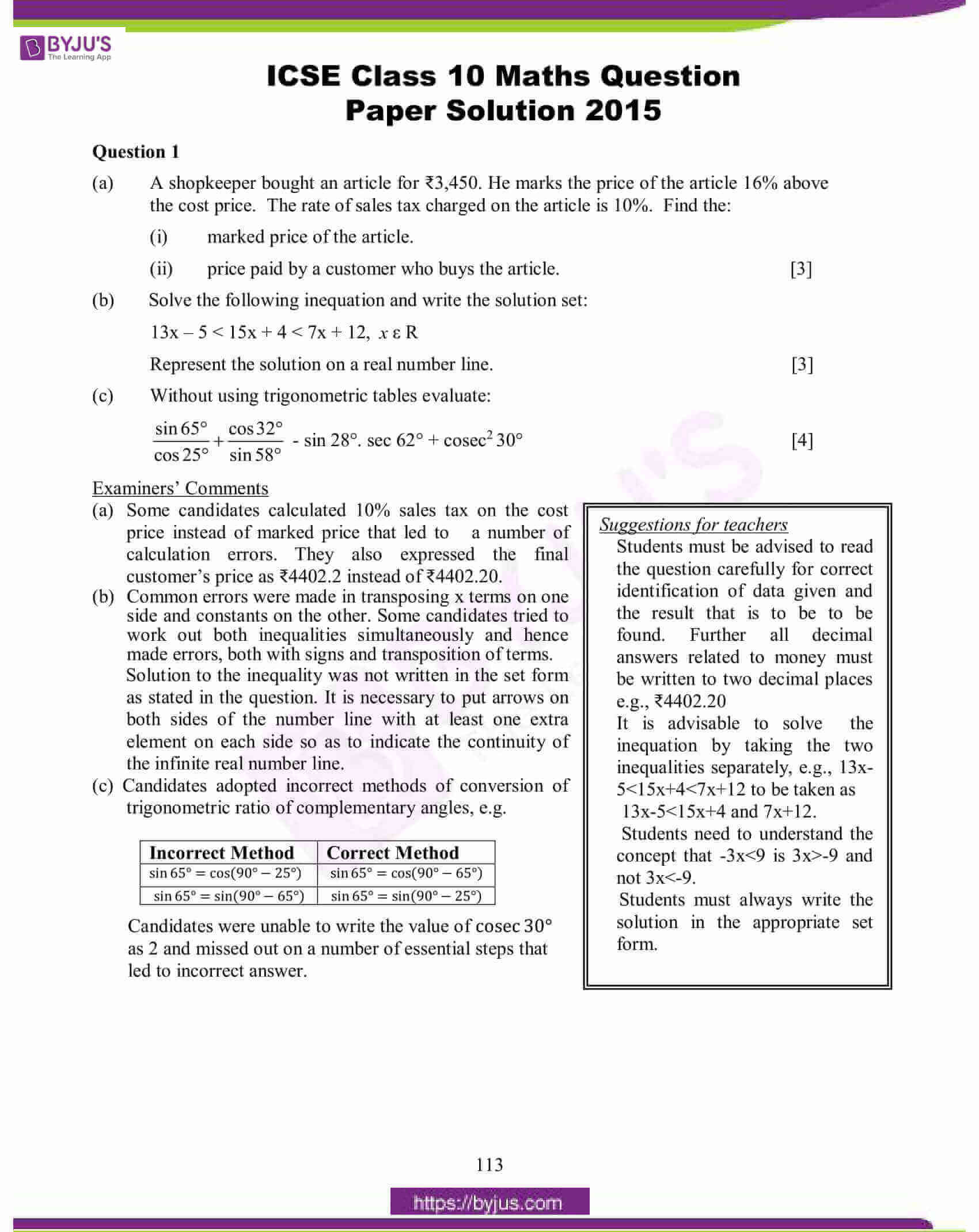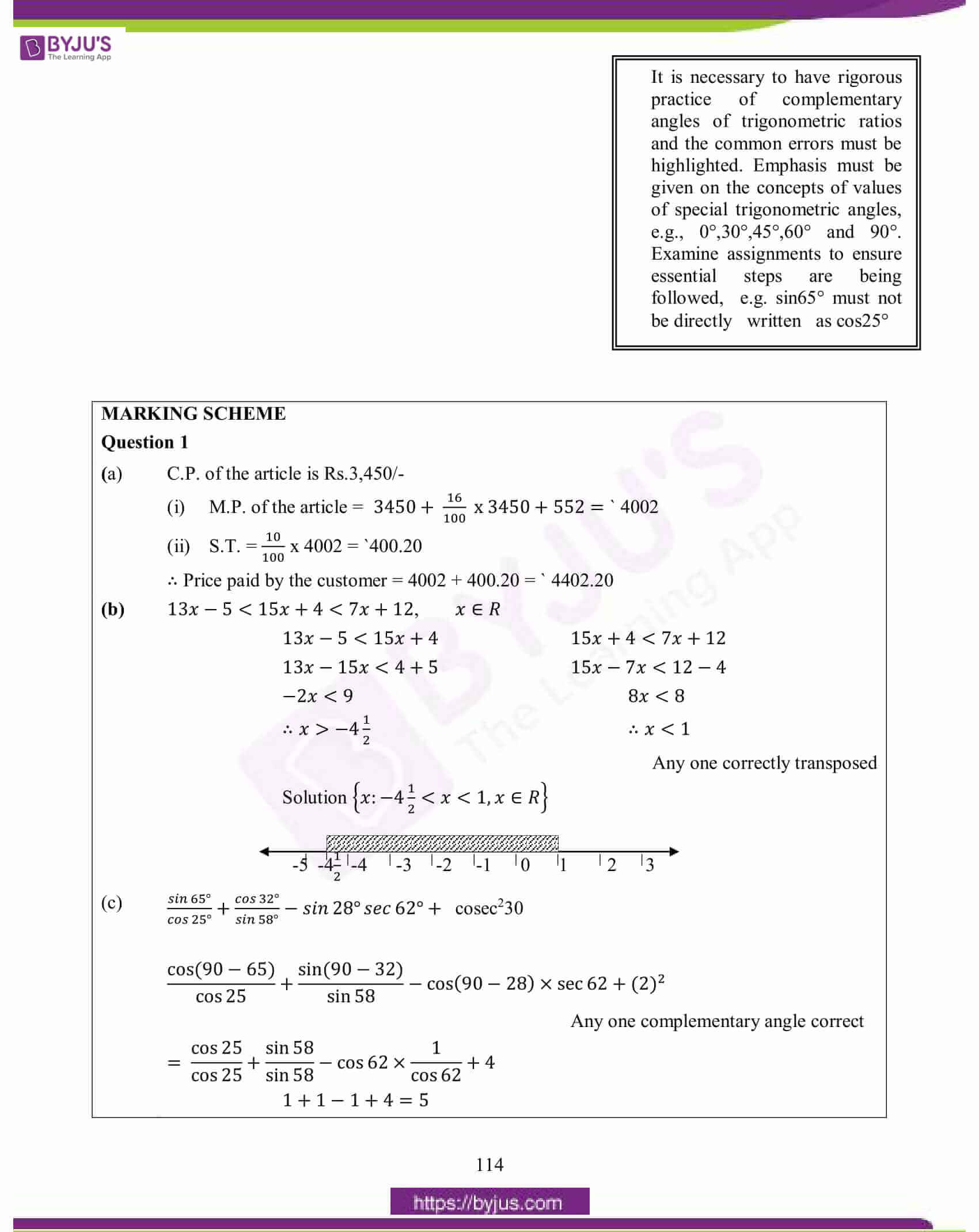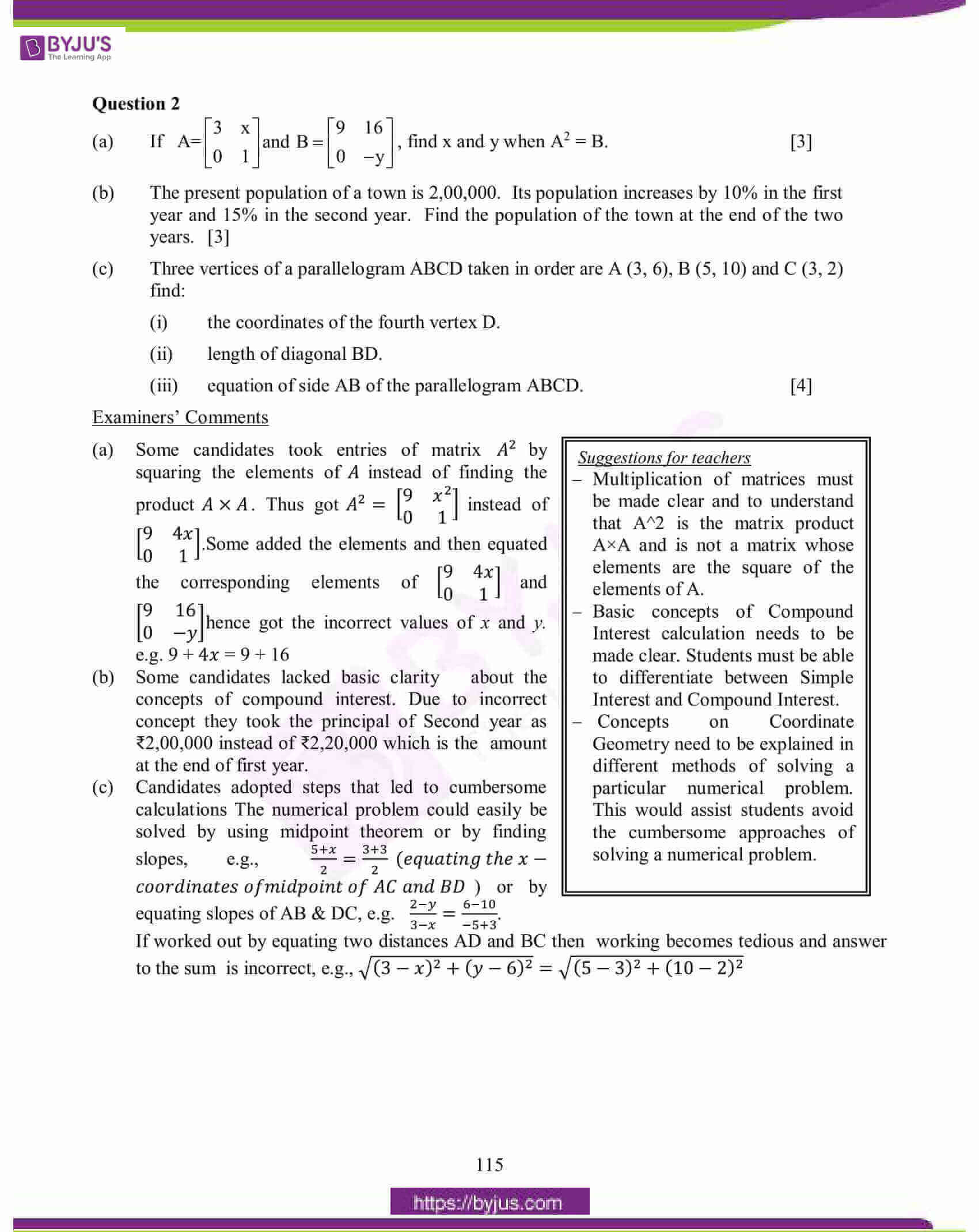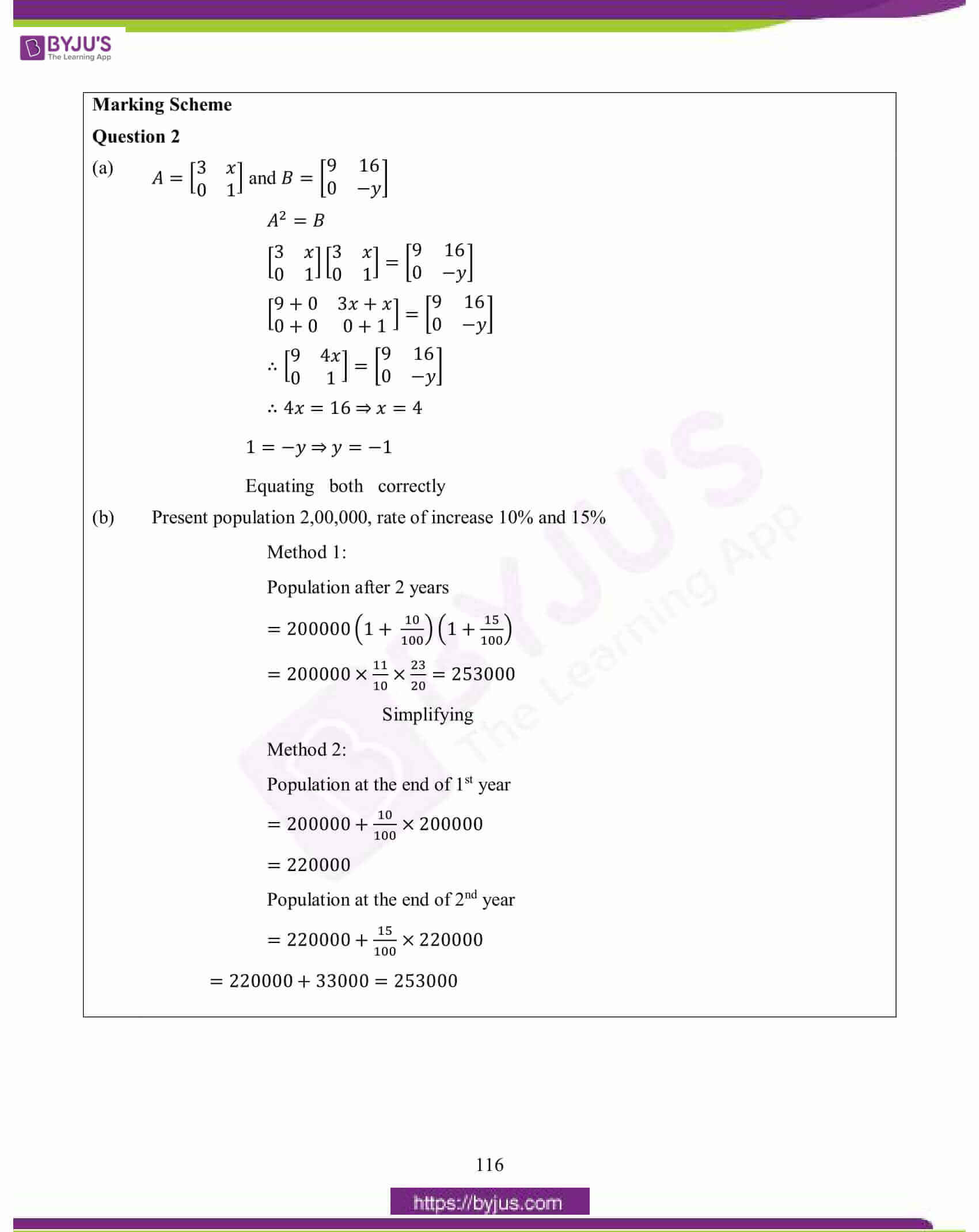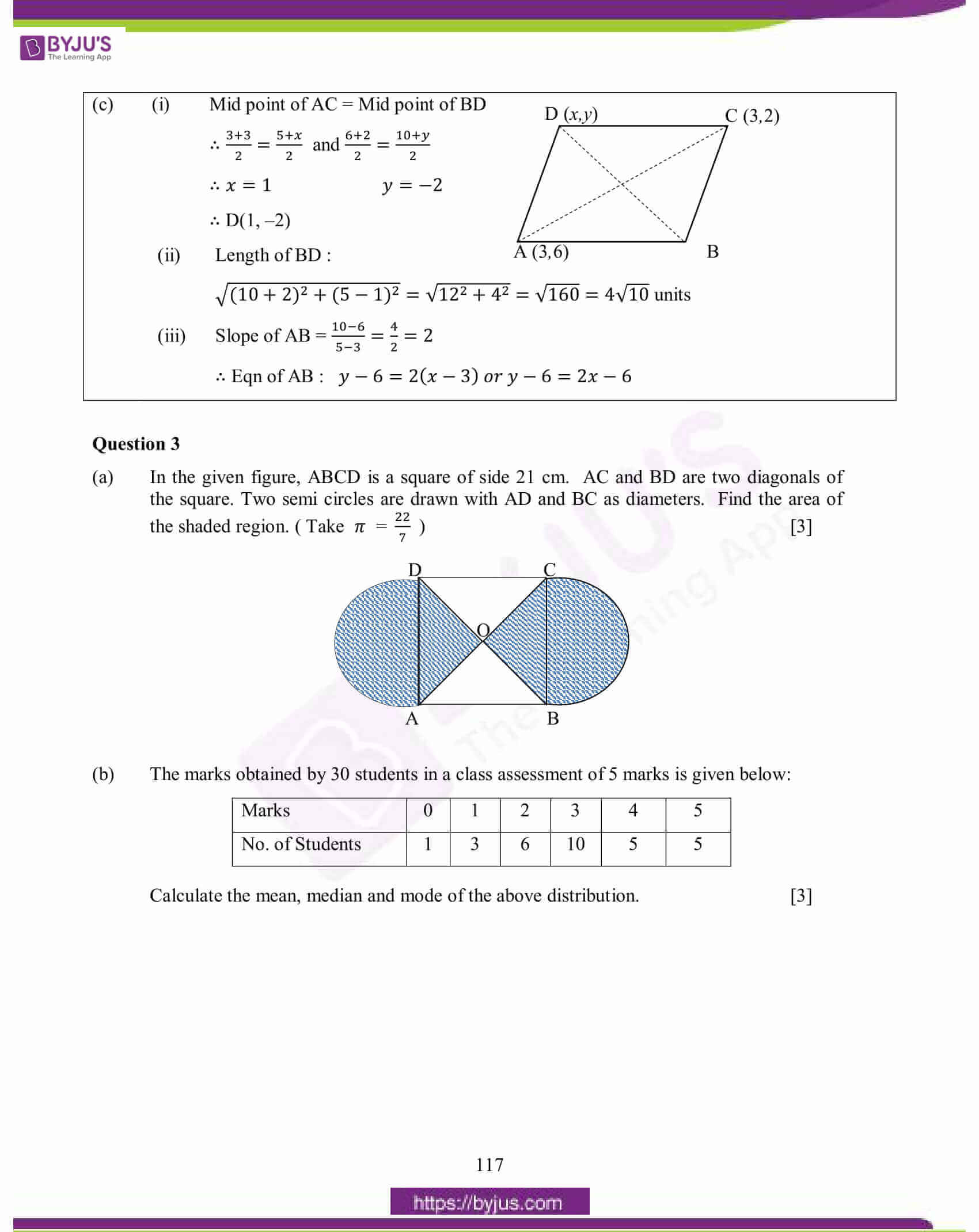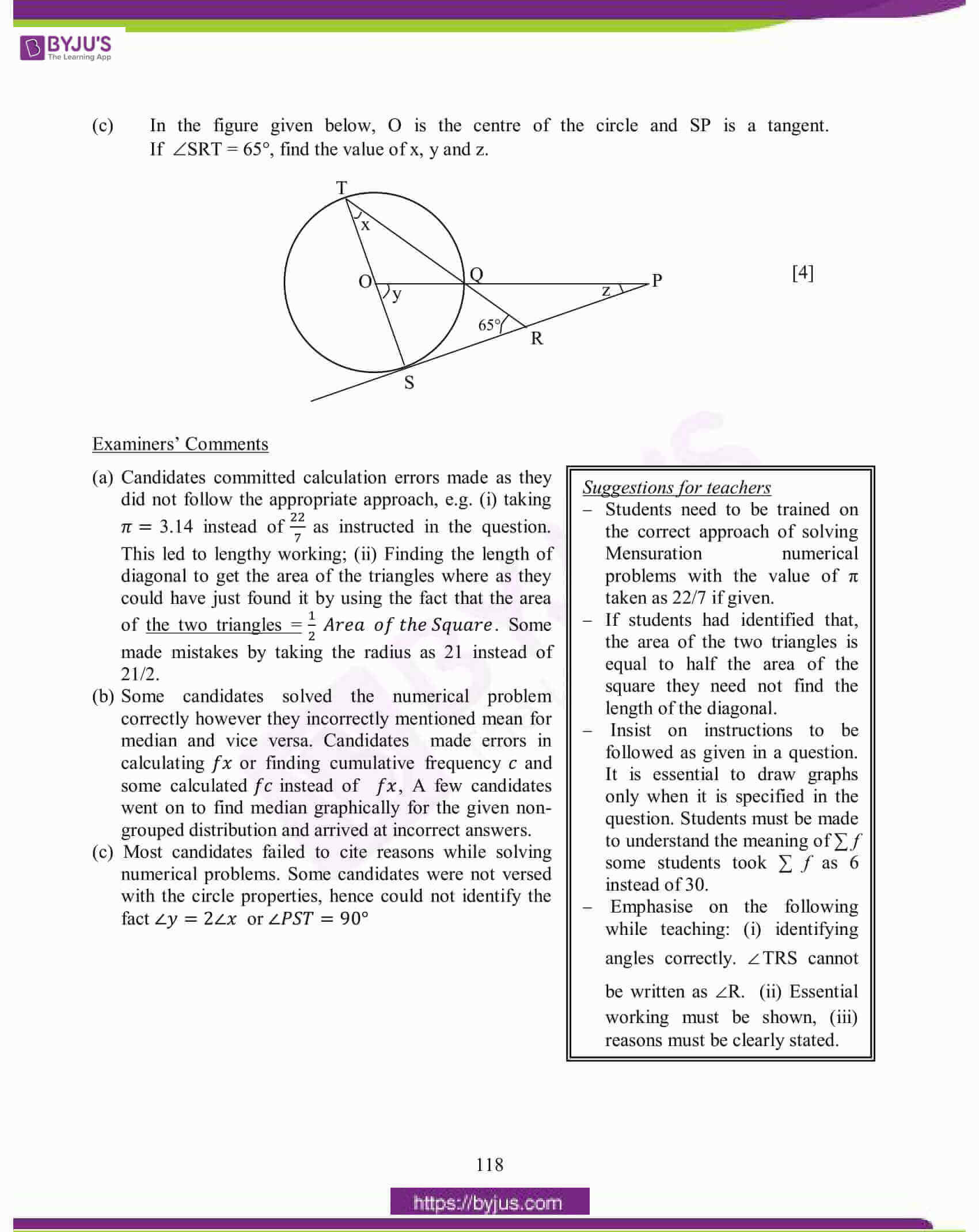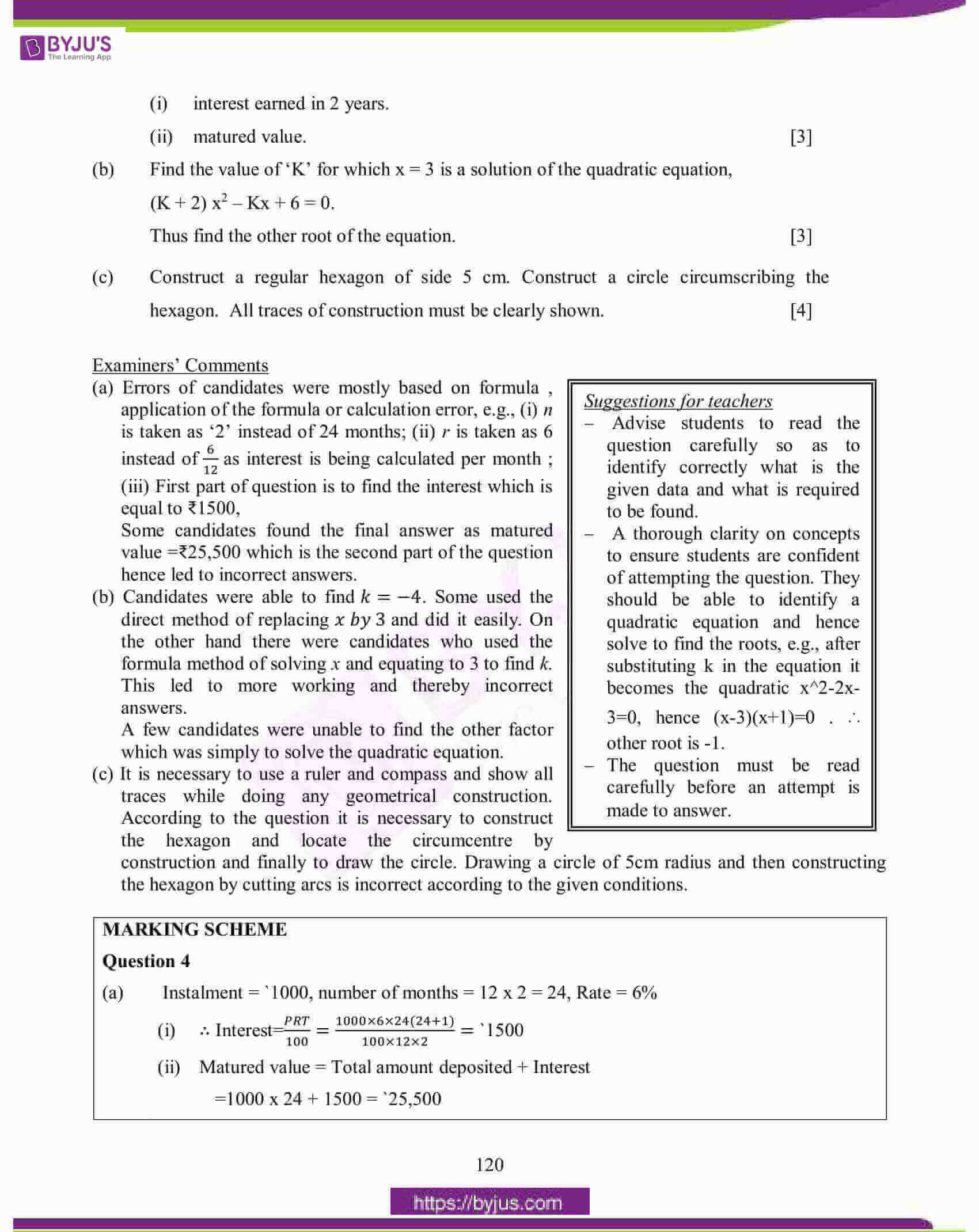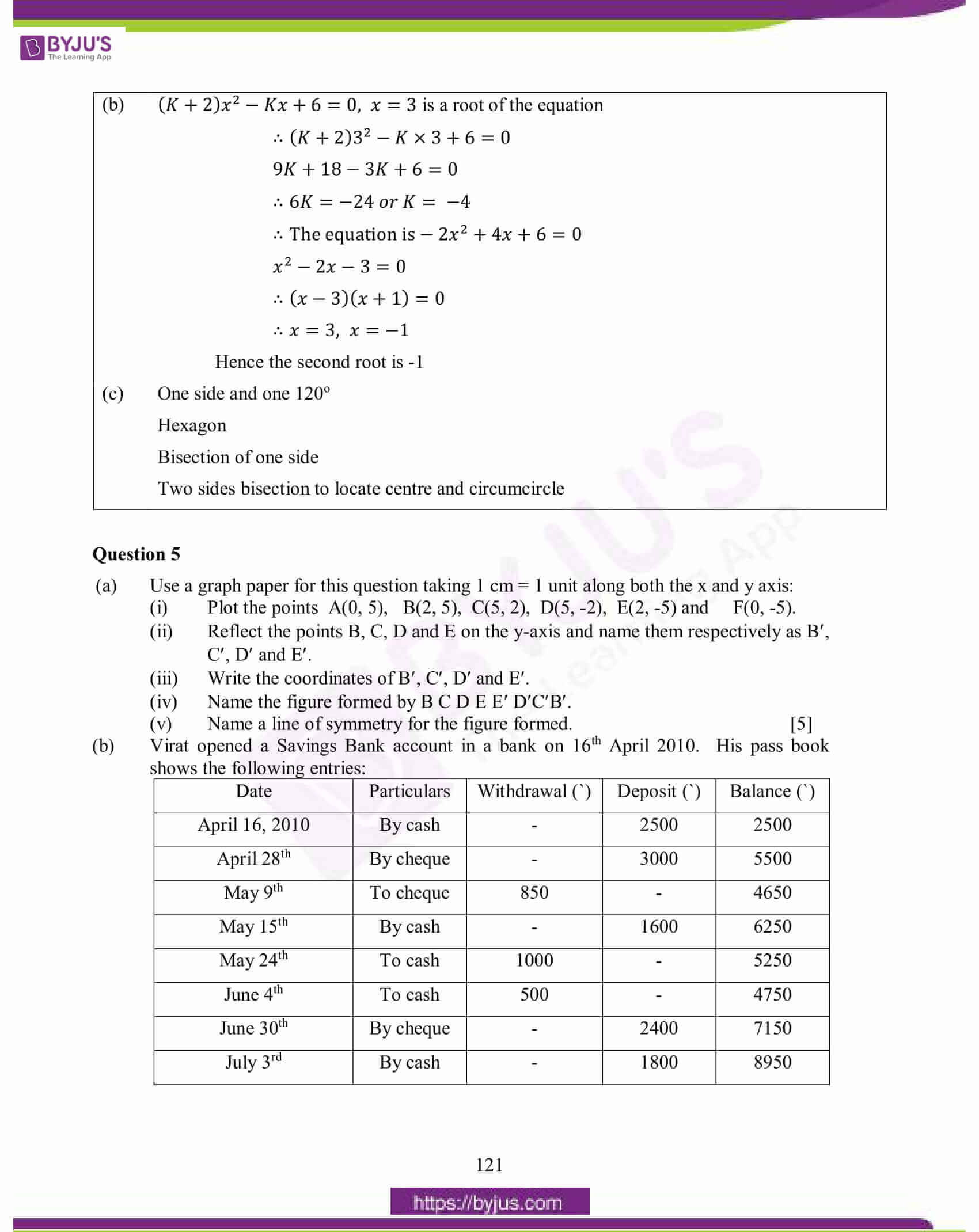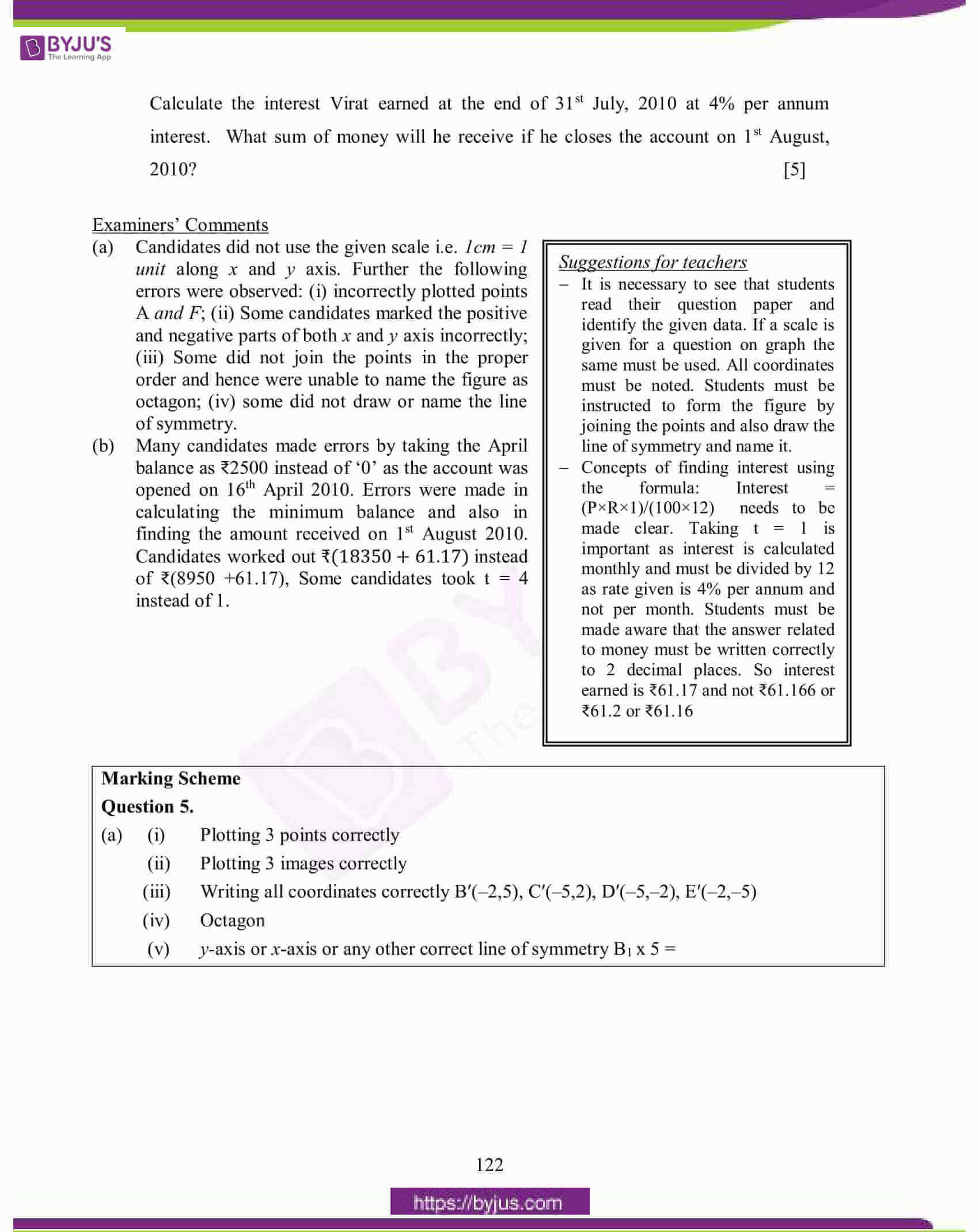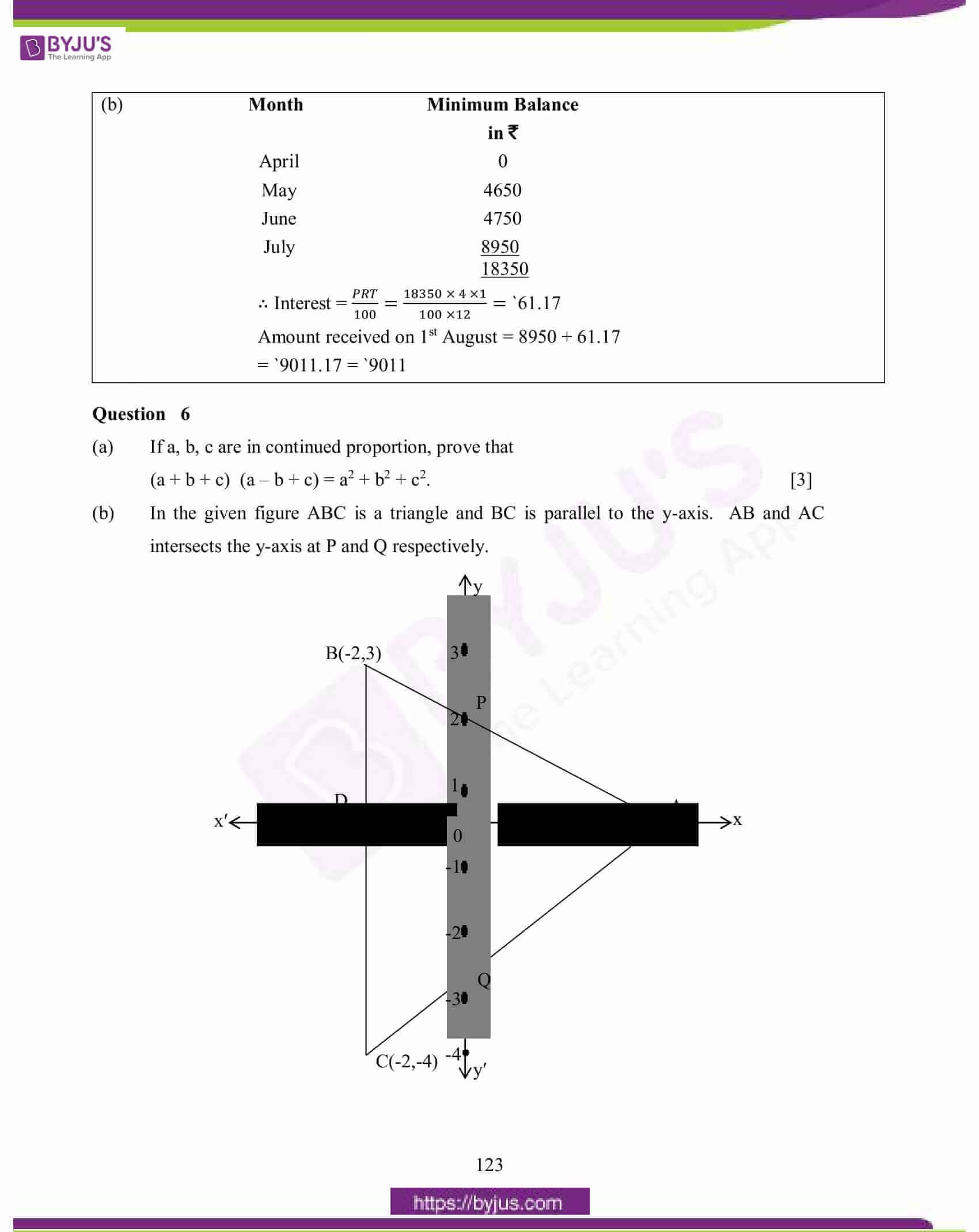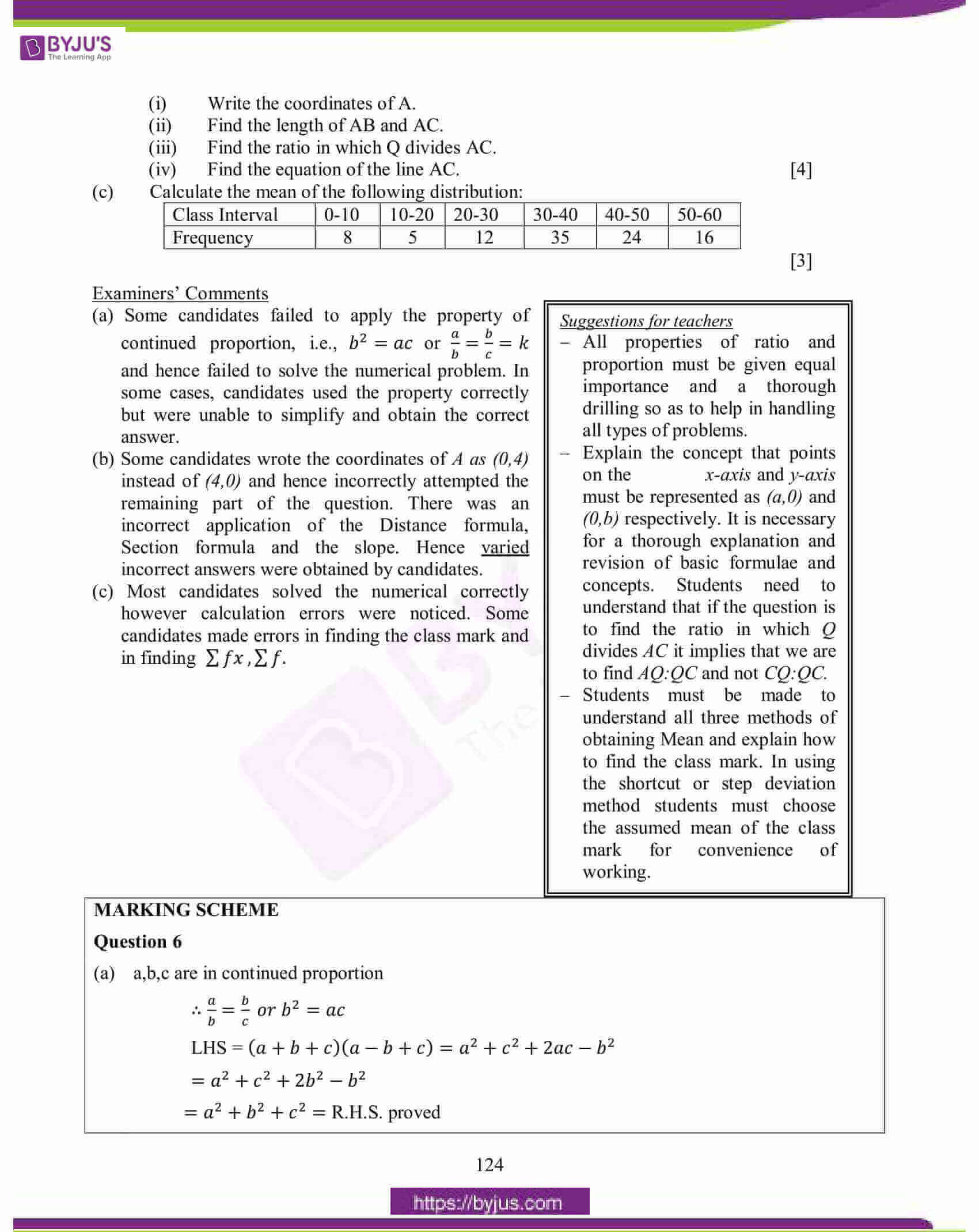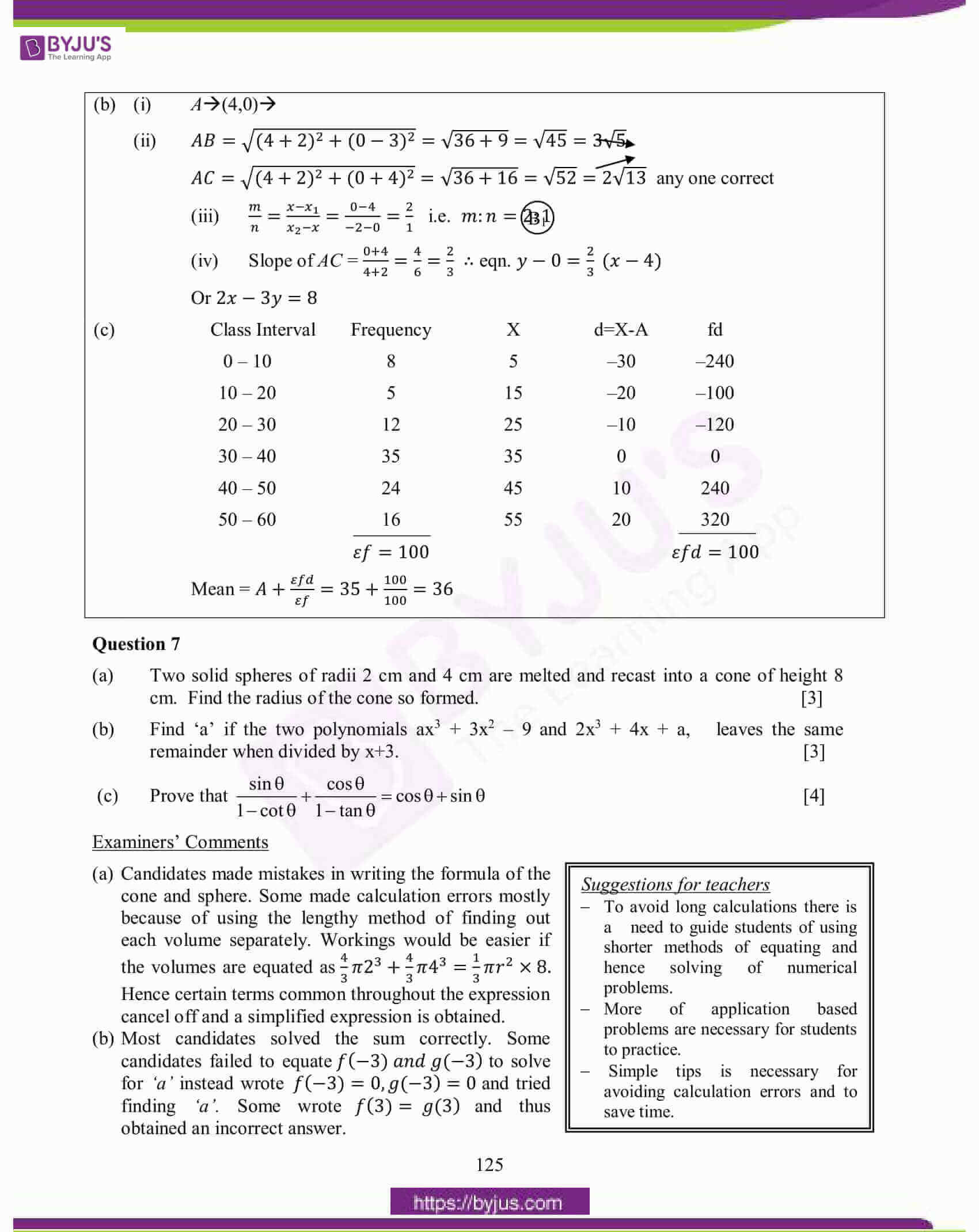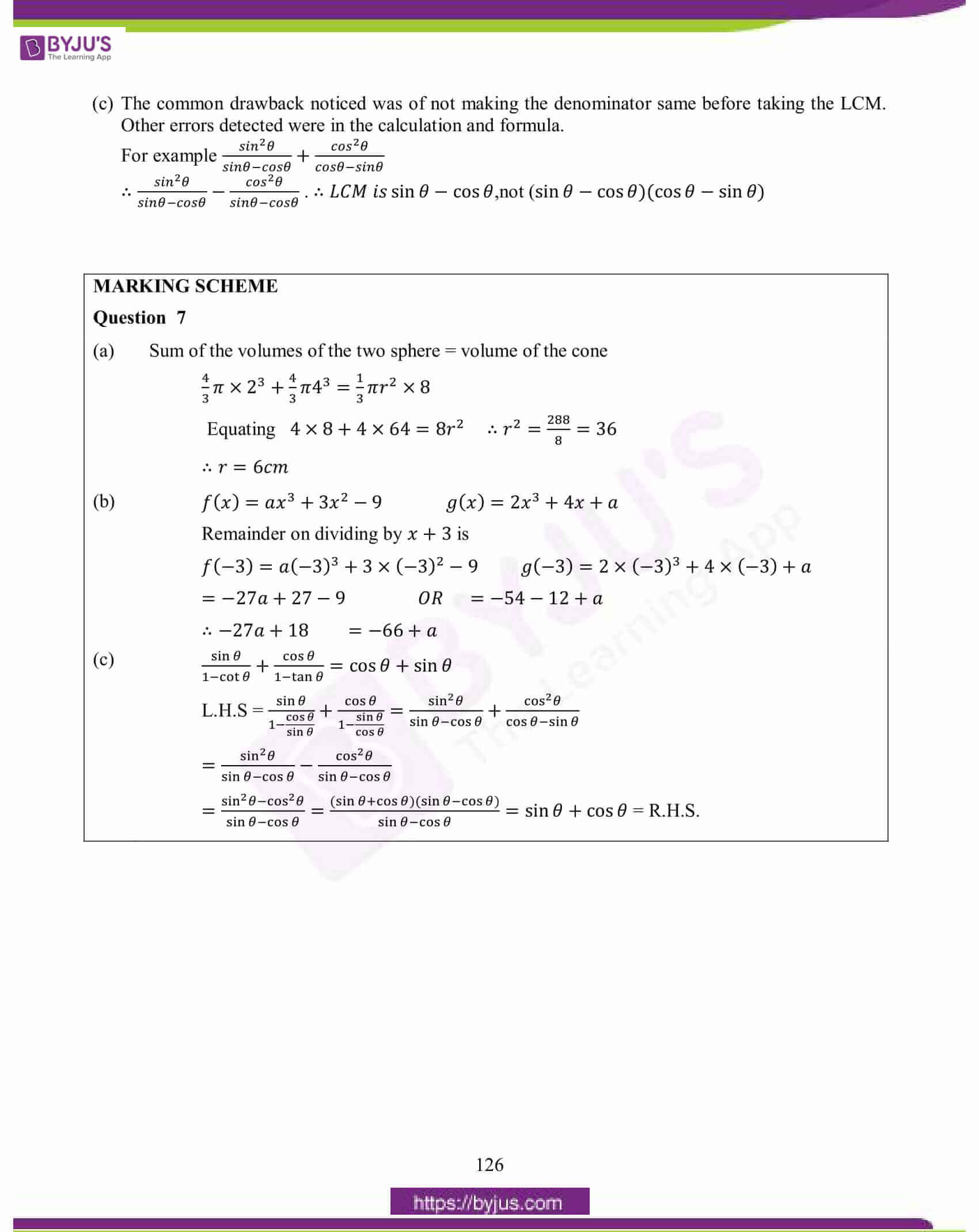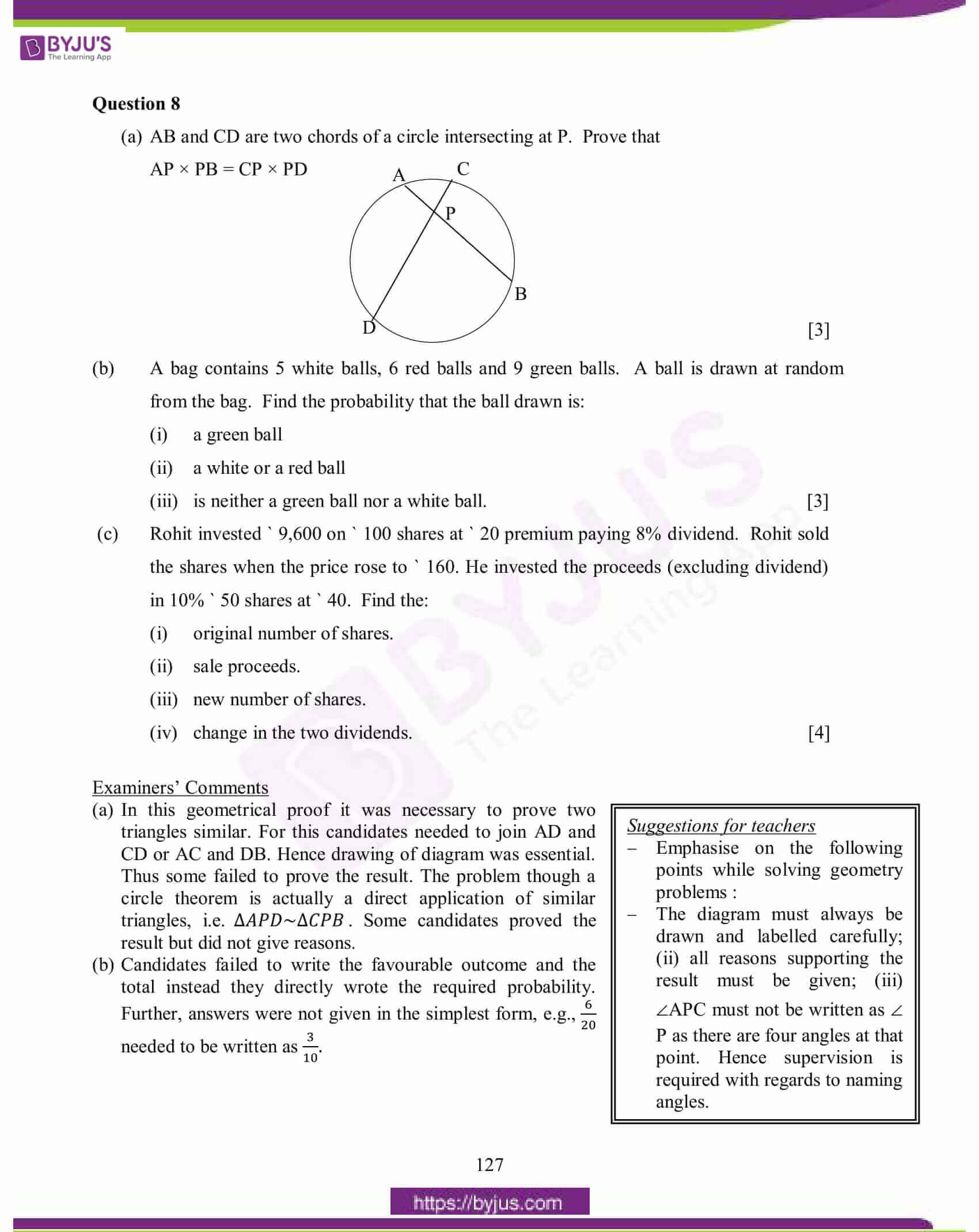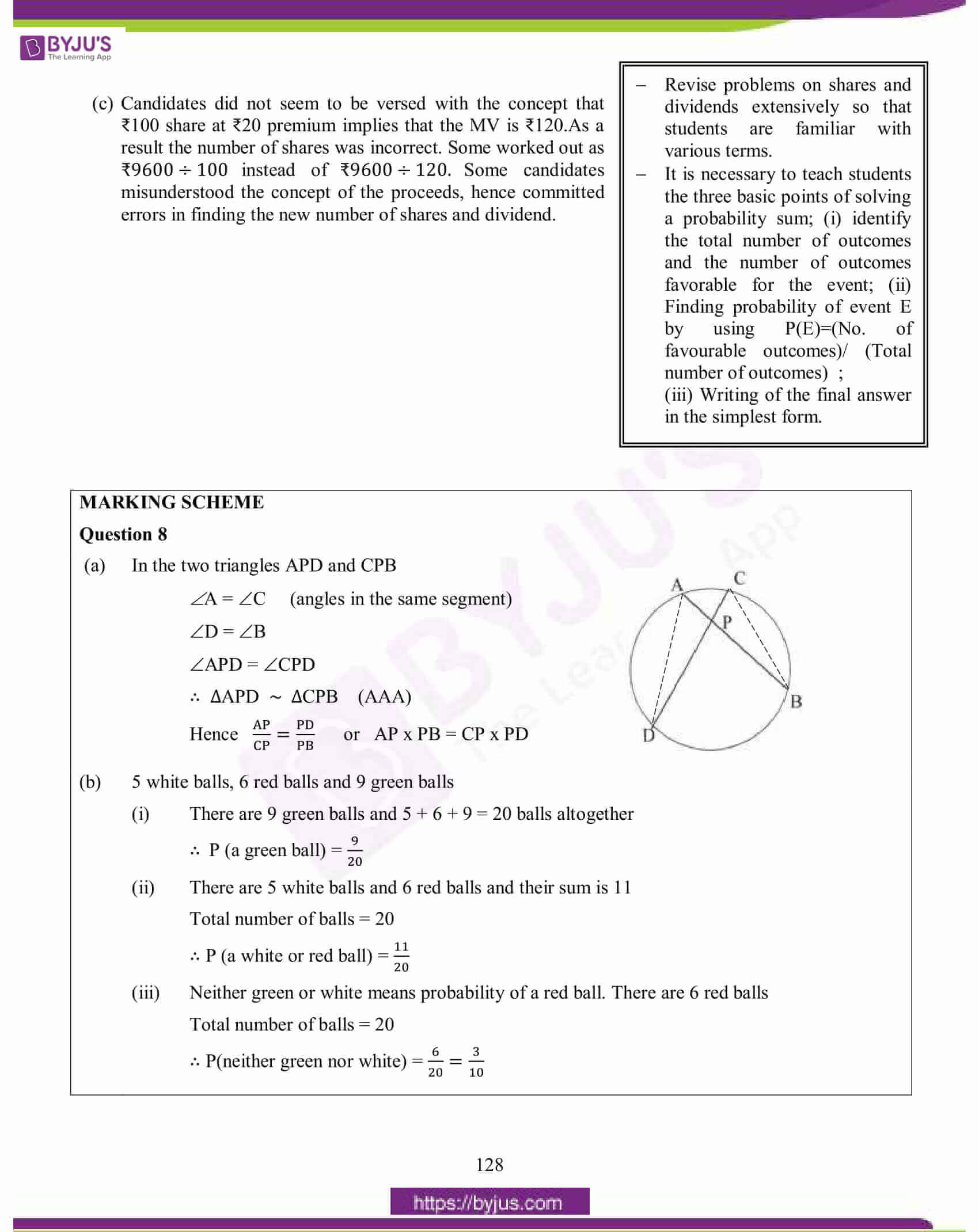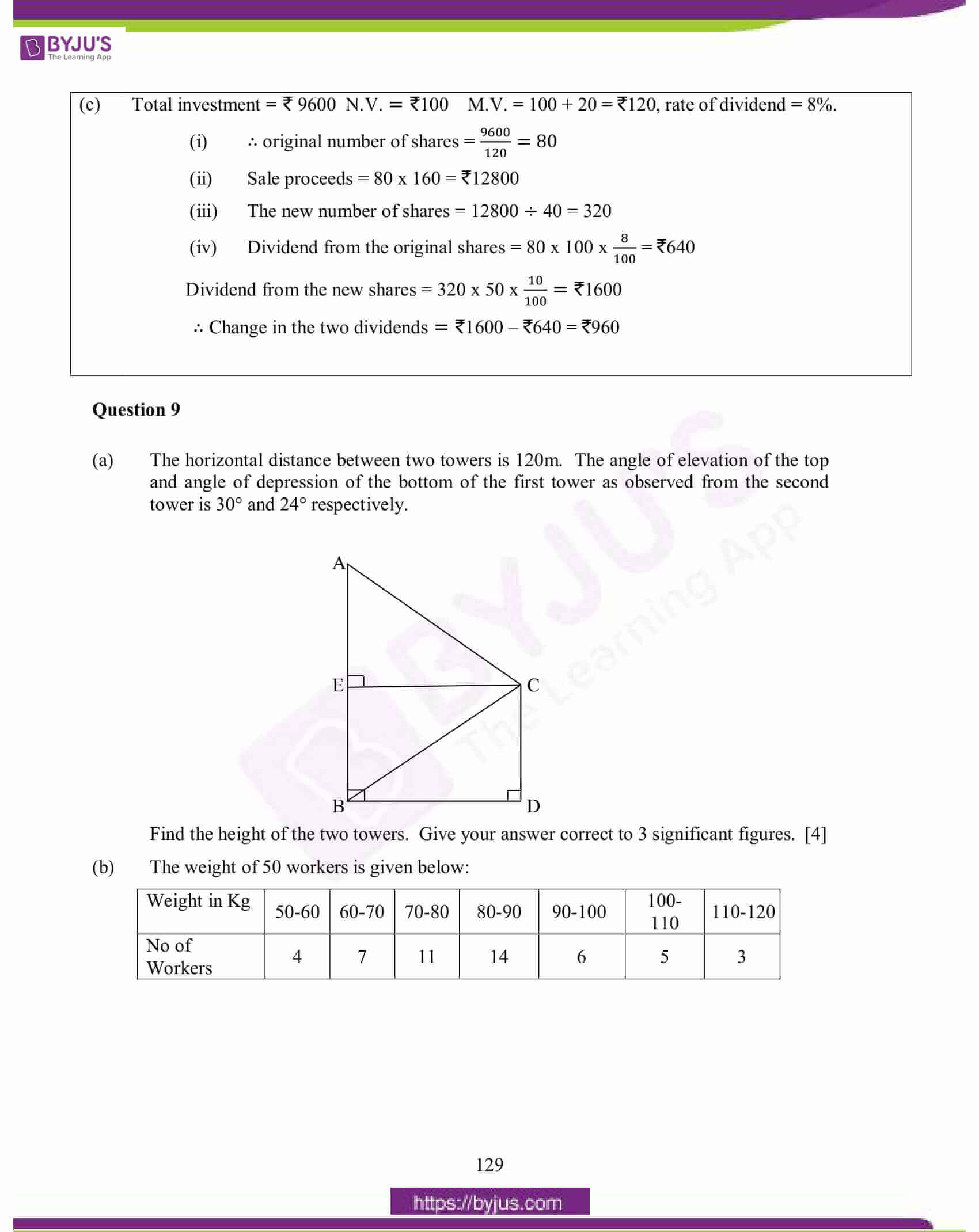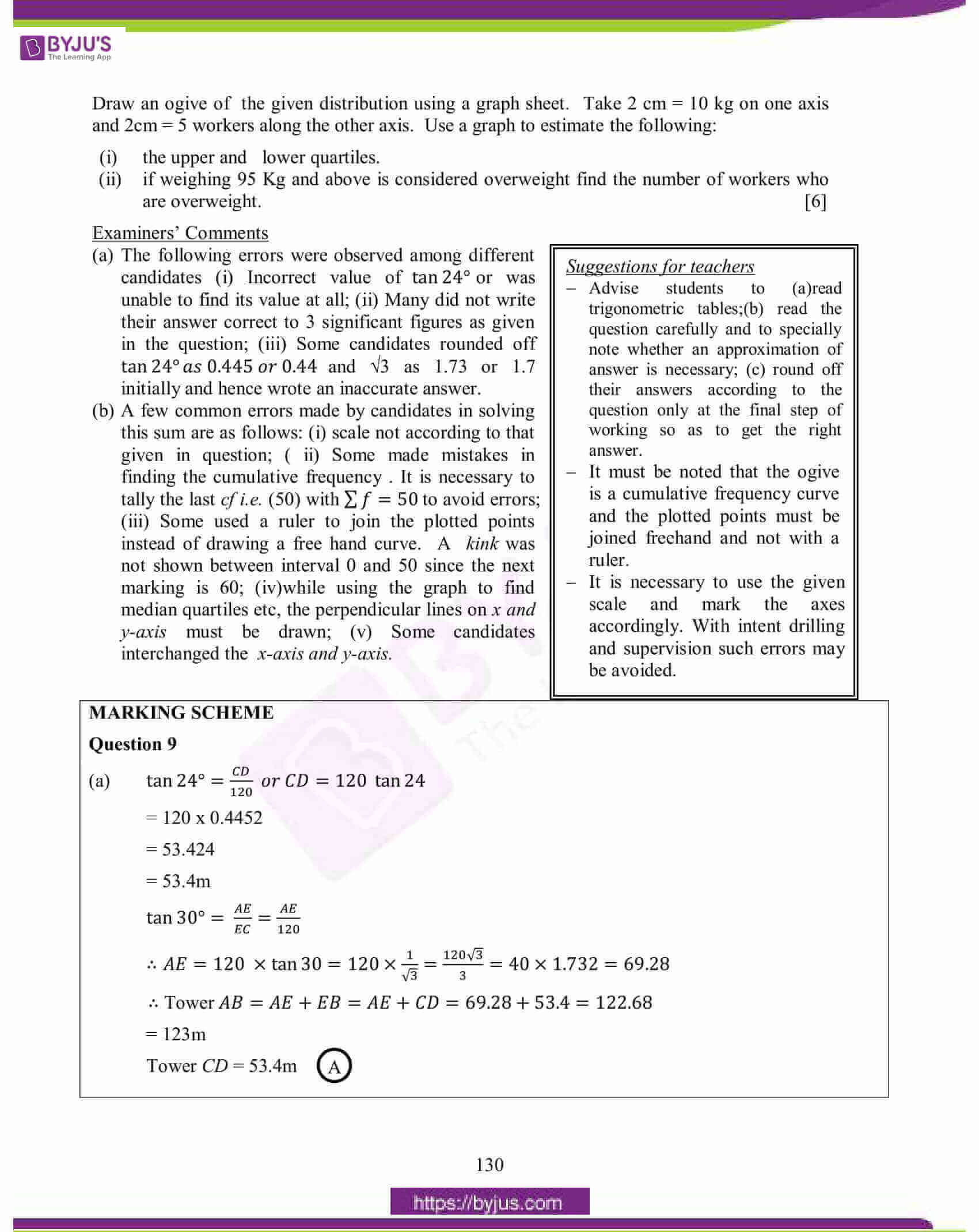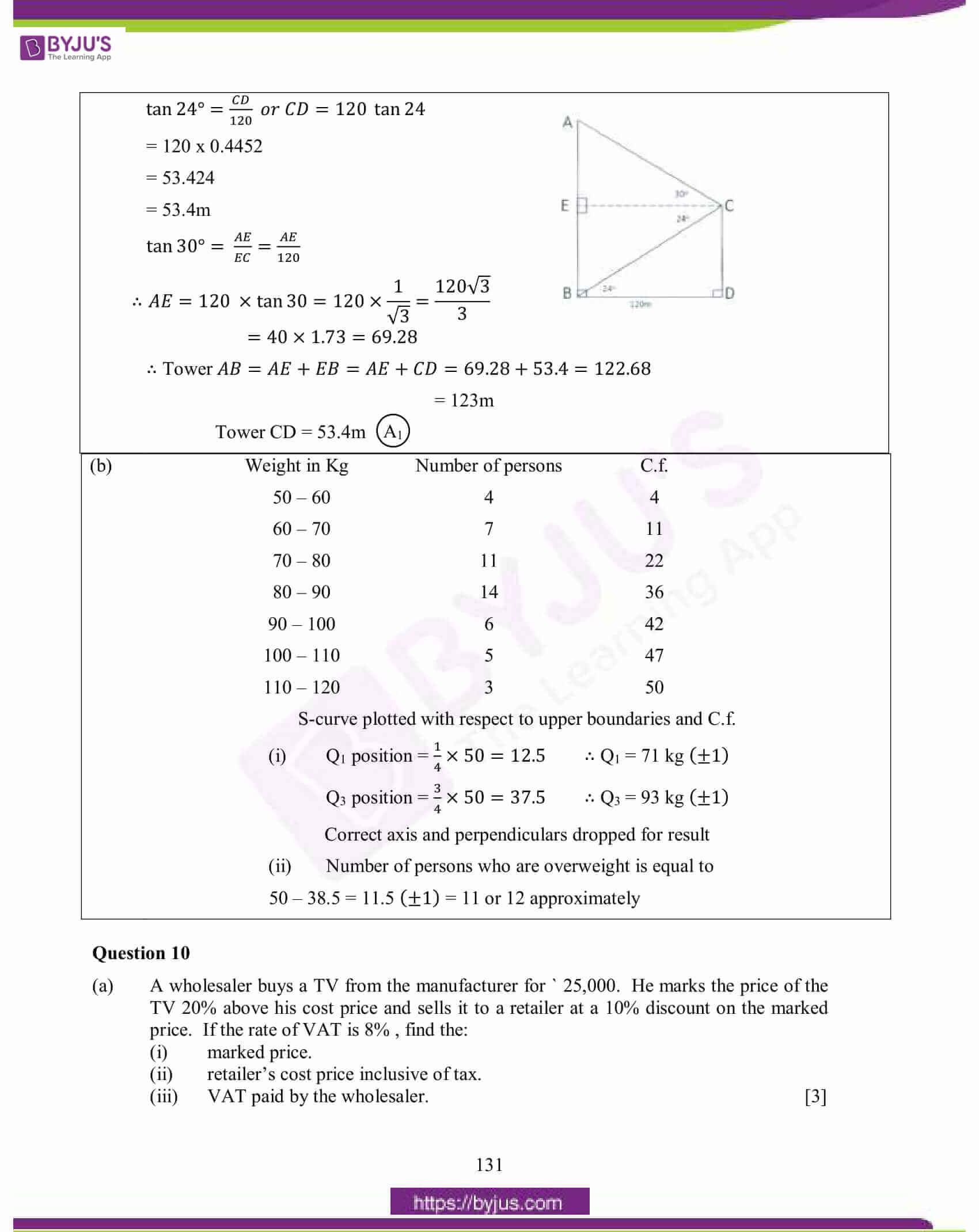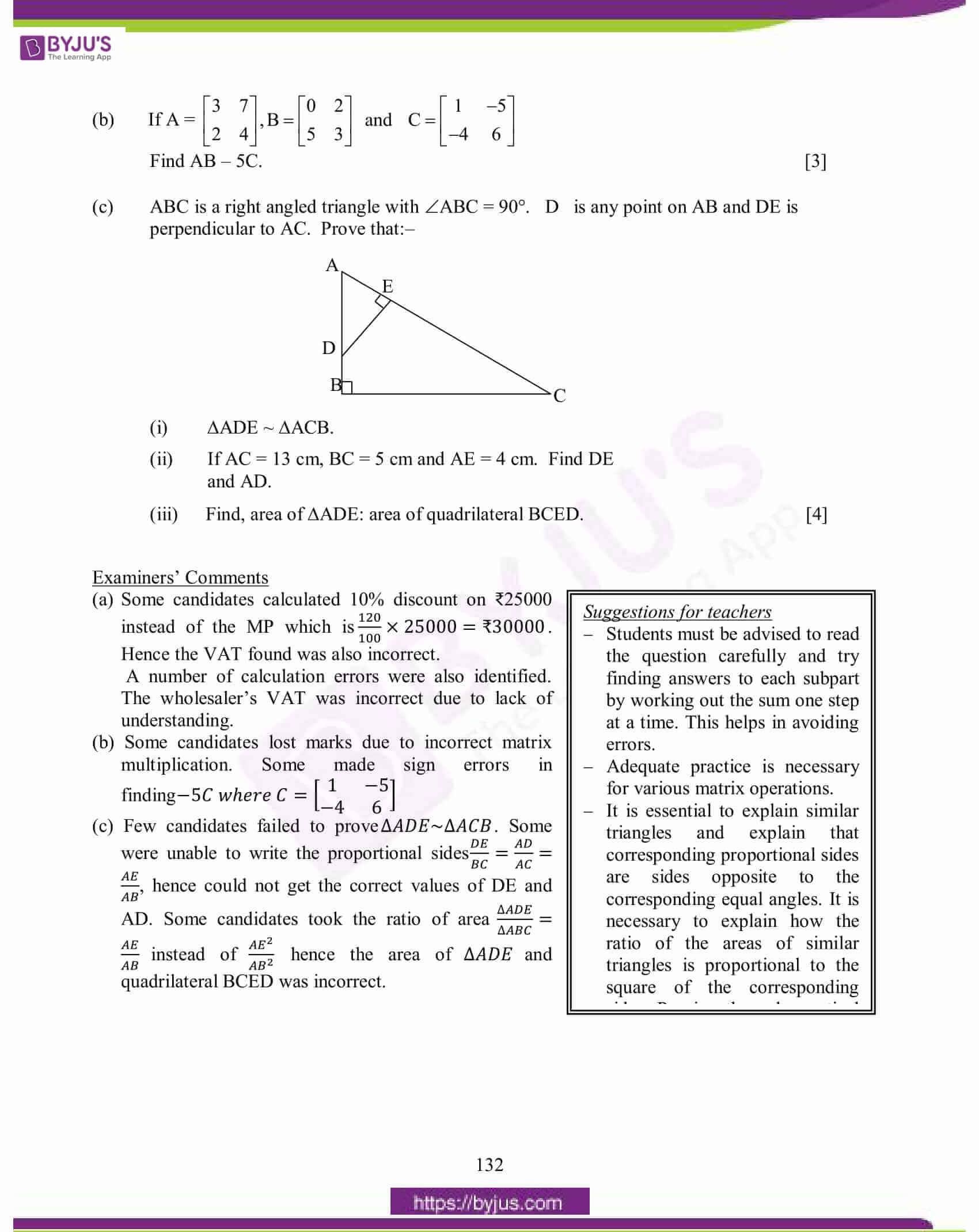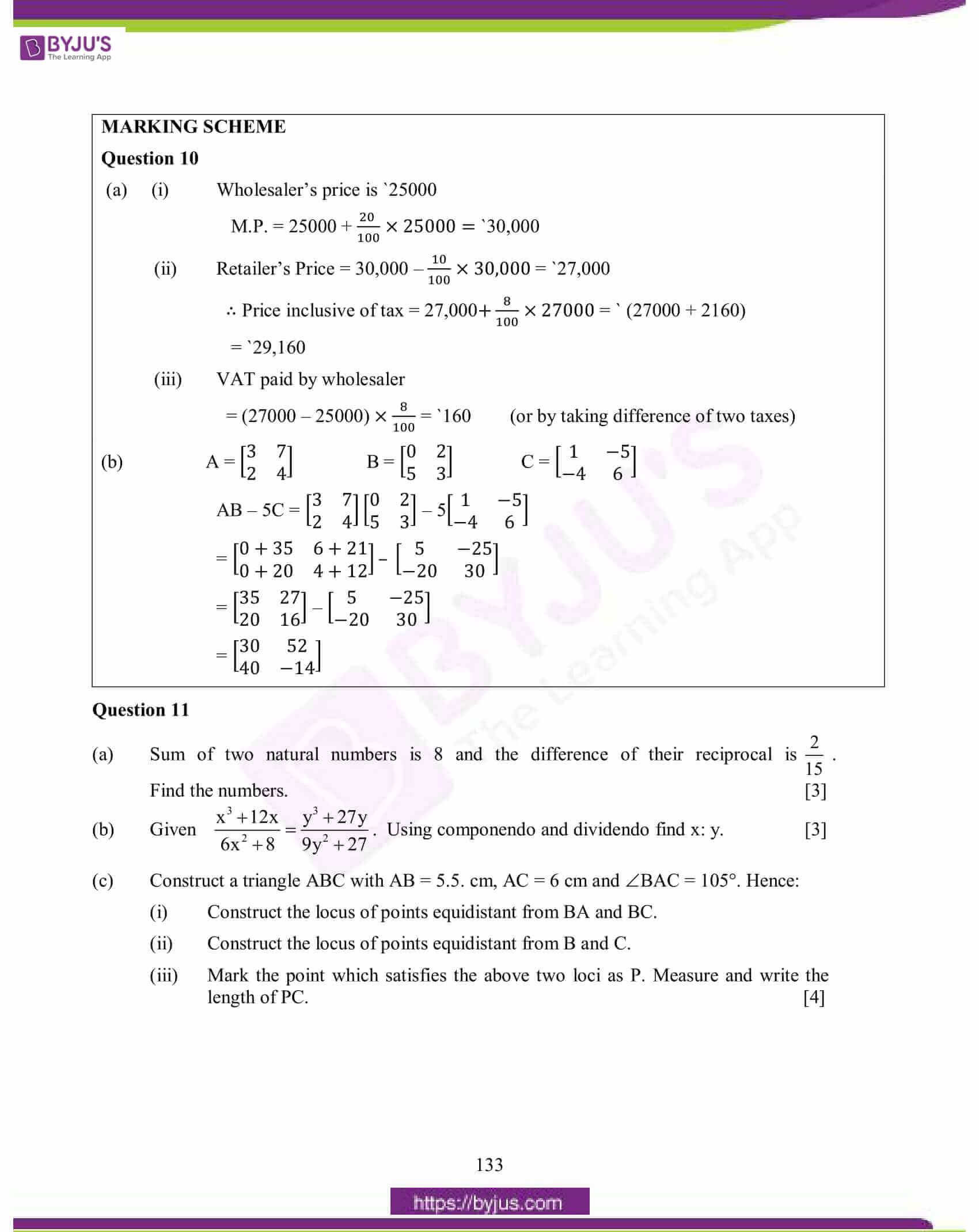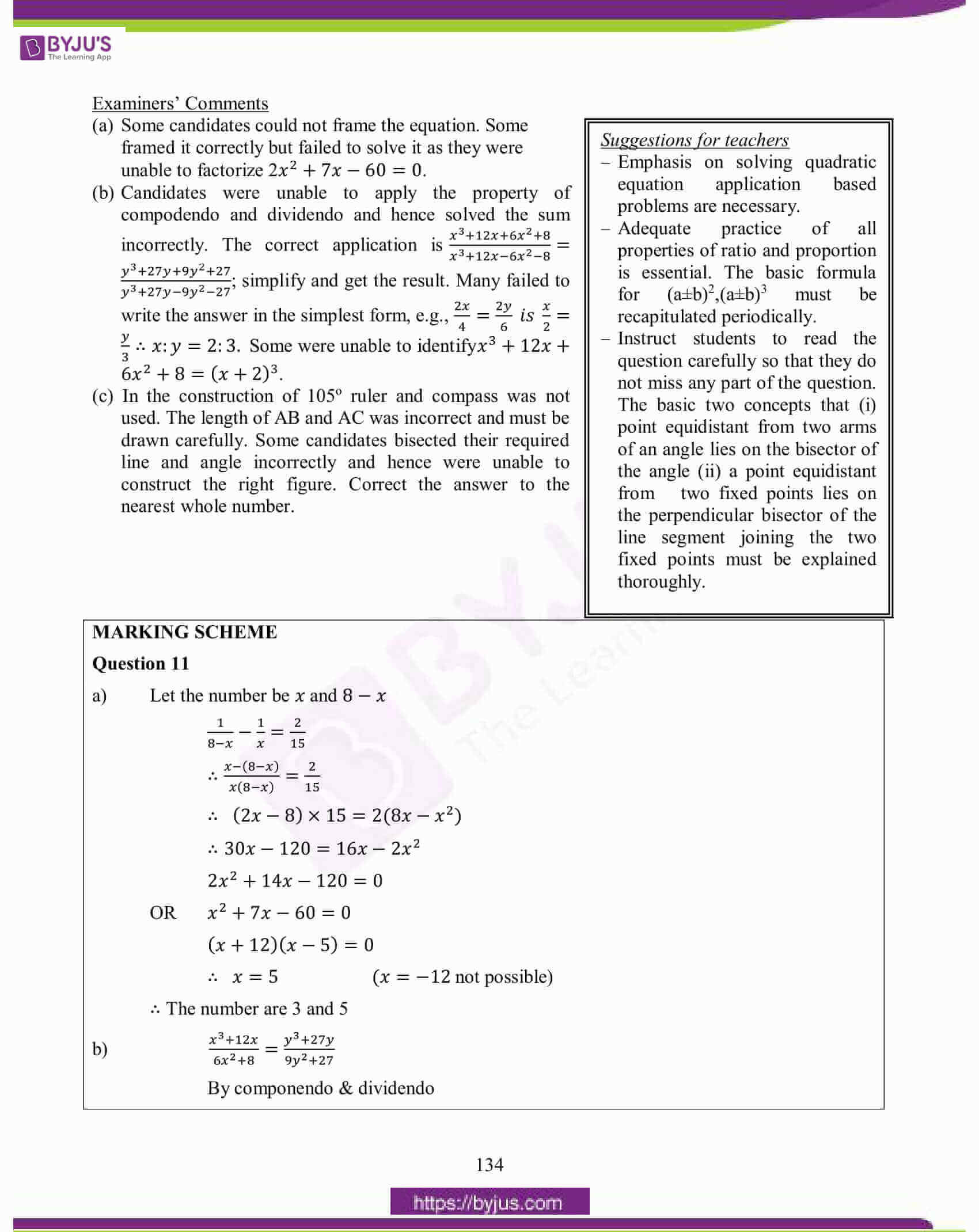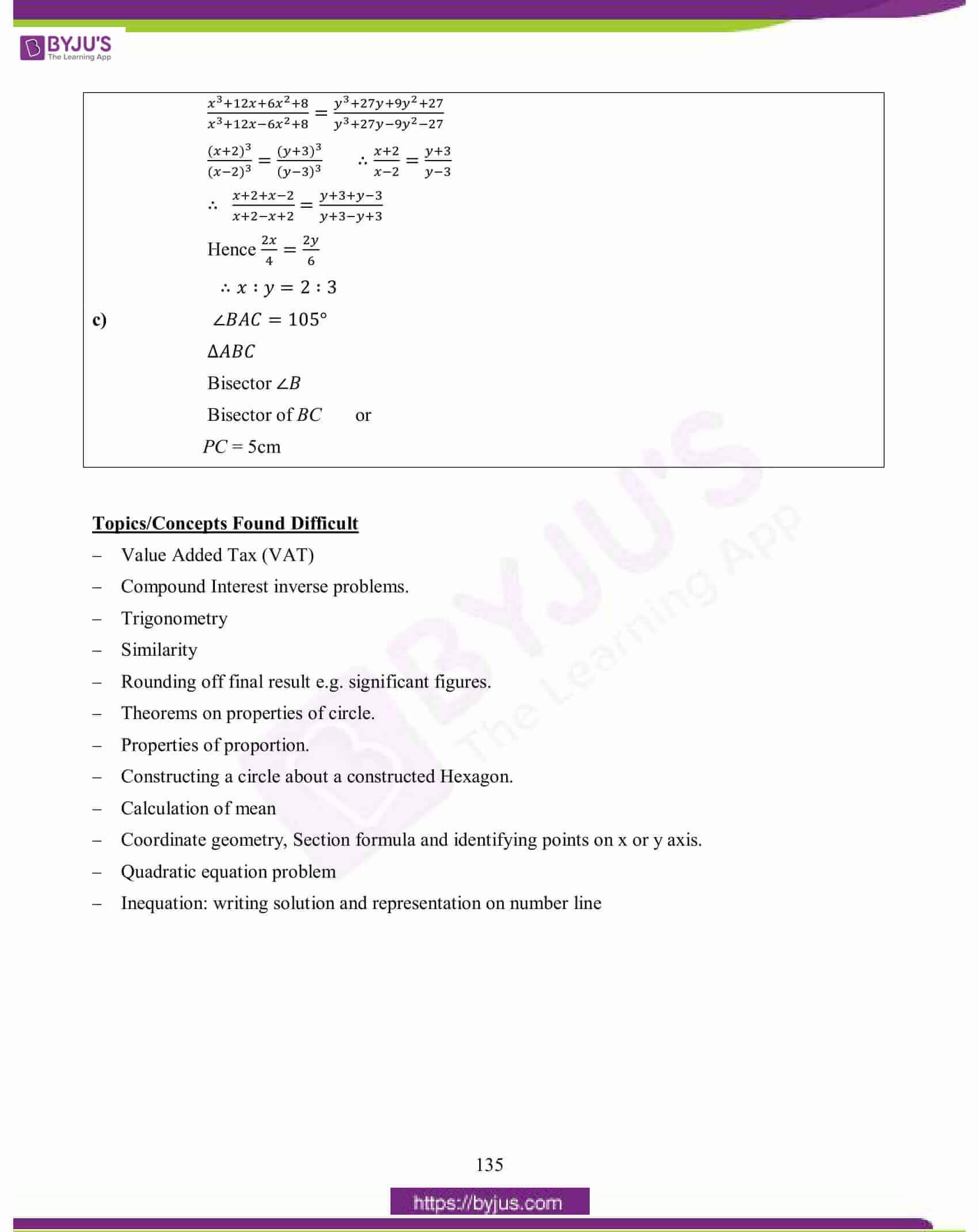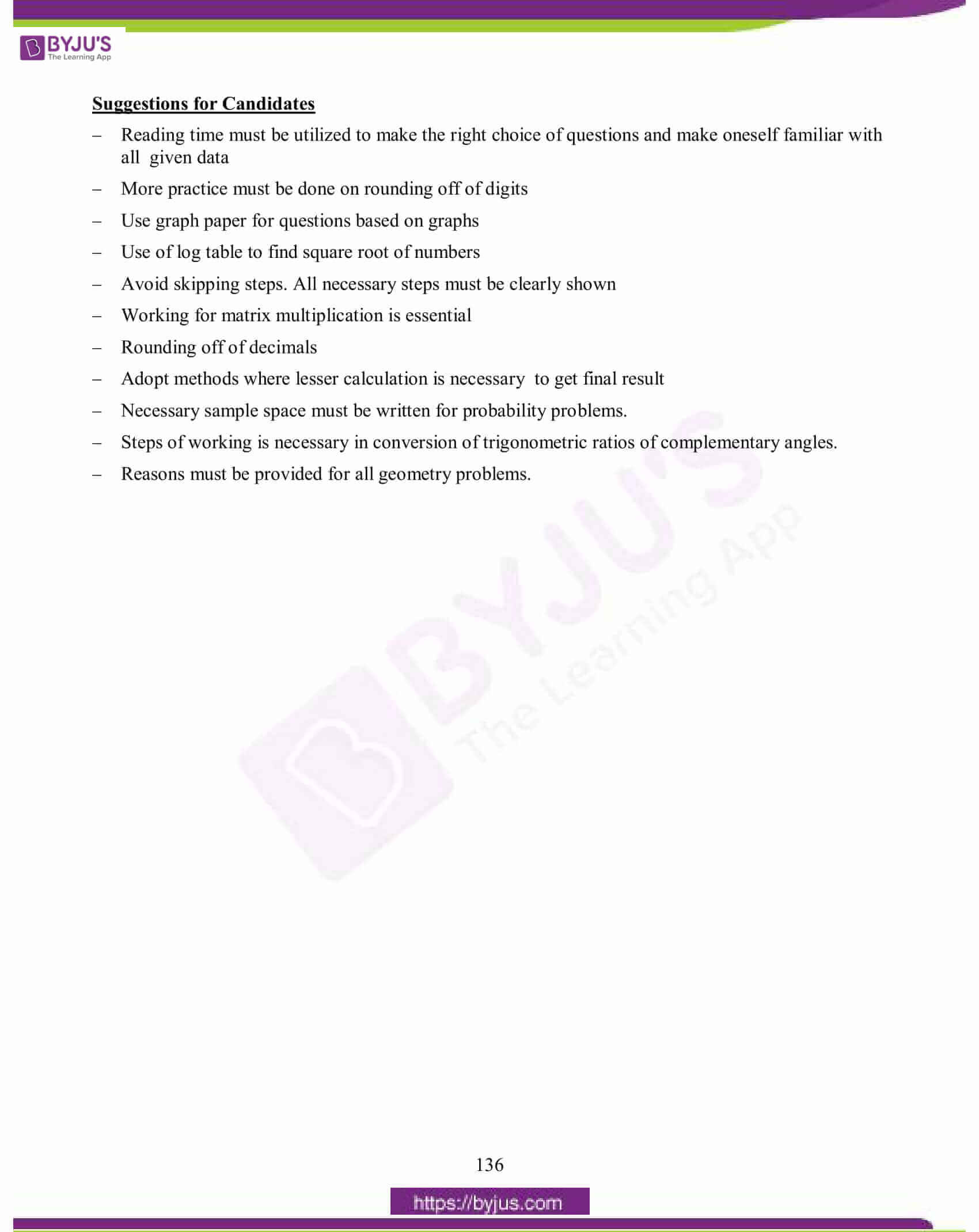### Topics Found Confusing and Difficult in Maths 2015 Question Paper

Here is the list of difficult and confusing topics of the 2015 Maths Paper which students found while attempting the paper.

• Compound Interest inverse problems.
• Trigonometry
• Similarity
• Rounding off final result e.g. significant figures.
• Theorems on properties of circles.
• Properties of proportion.
• Constructing a circle about a constructed Hexagon.
• Calculation of mean
• Coordinate geometry, Section formula and identifying points on the x or y axis.
• Inequation: writing solution and representation on number line

## ICSE Class 10 Maths Question Paper 2015 With Solution

### Question 1:

(a) A shopkeeper bought an article for ₹3,450. He marks the price of the article 16% above the cost price. The rate of sales tax charged on the article is 10%. Find the:

(i) marked price of the article.

(ii) price paid by a customer who buys the article.

(b) Solve the following inequation and write the solution set:

13x – 5 < 15x + 4 < 7x + 12, 𝑥∈𝑅

Represent the solution on a real number line.

(c) Without using trigonometric tables evaluate: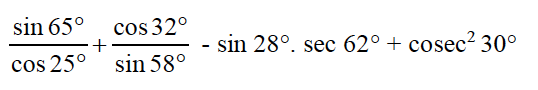(a) C.P. of the article is Rs.3,450/-

(i) M.P. of the article = 3450 + (16/100)x3450 + 552 = 4002

(ii) S.T. = (10/100)x4002 = 400.20

∴ Price paid by the customer = 4002 + 400.20 = 4402.20

(b) 13𝑥 − 5 < 15𝑥 + 4 < 7𝑥 + 12, 𝑥∈𝑅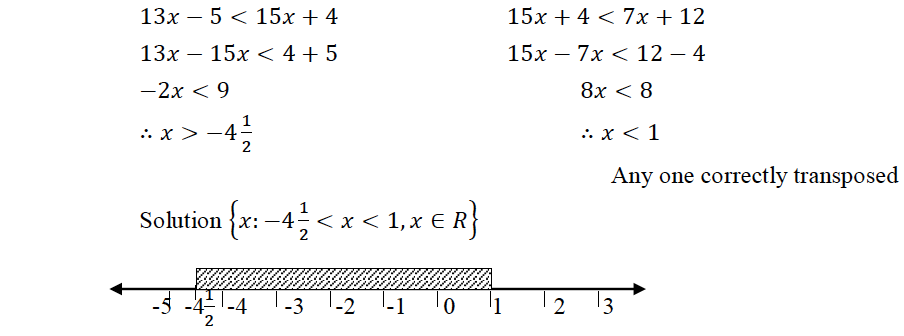(c)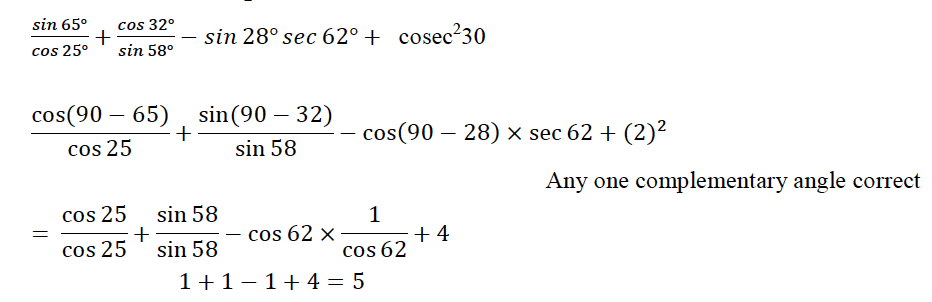### Question 2:

(a)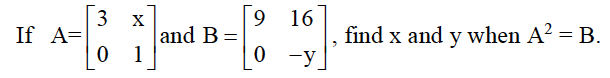(b) The present population of a town is 2,00,000. Its population increases by 10% in the first year and 15% in the second year. Find the population of the town at the end of the two years.

(c) Three vertices of a parallelogram ABCD taken in order are A (3, 6), B (5, 10) and C (3, 2) find:

(i) the coordinates of the fourth vertex D.

(ii) length of diagonal BD.

(iii) equation of side AB of the parallelogram ABCD.

(a)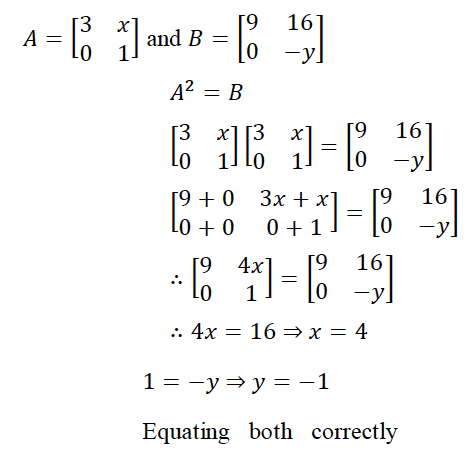(b) Present population 2,00,000, rate of increase 10% and 15%

Method 1:

Population after 2 years

= 200000(1+ 10/100)(1+ 15/100)

= 200000 × 11/10 × 23/20 = 253000

Simplifying

Method 2:

Population at the end of 1st year

= 200000 + 10/100 × 200000

= 220000

Population at the end of 2nd year

= 220000 + 15/100 × 220000

= 220000 + 33000 = 253000

(c)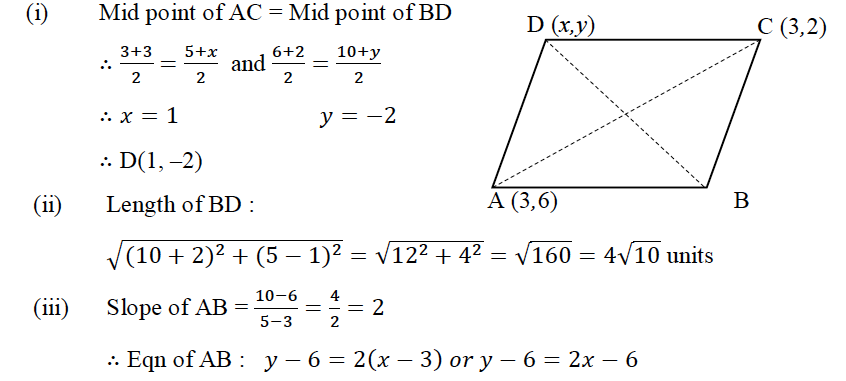### Question 3:

(a) In the given figure, ABCD is a square of side 21 cm. AC and BD are two diagonals of the square. Two semi circles are drawn with AD and BC as diameters. Find the area of the shaded region. ( Take 𝜋 = 22/7 )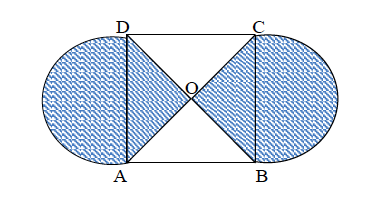(b) The marks obtained by 30 students in a class assessment of 5 marks is given below:

 Marks 0 1 2 3 4 5 No. of Students 1 3 6 10 5 5

Calculate the mean, median and mode of the above distribution.

(c) In the figure given below, O is the centre of the circle and SP is a tangent. If SRT = 65°, find the value of x, y and z.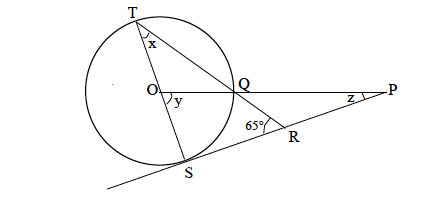(a)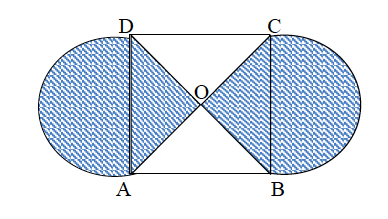ABCD is a square of side 21 cm.

∴ Area of square = 212 = 441

∴ Area of the shaded part = area of the two triangular part + Area of the two semi circles.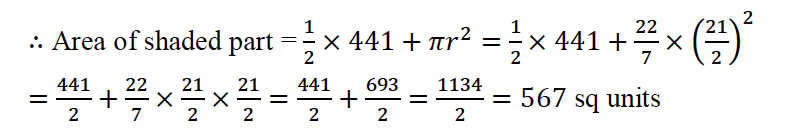(b)

 Marks (x) No. of Students (f) fx cf 0 1 0 1 1 3 3 4 2 6 12 10 3 10 30 20 4 5 20 25 5 5 25 30 30 90

∴ Mean = 𝜀𝑓𝑥 /𝜀𝑓 = 90/30 = 3

Median = 3

Mode = 3

(c)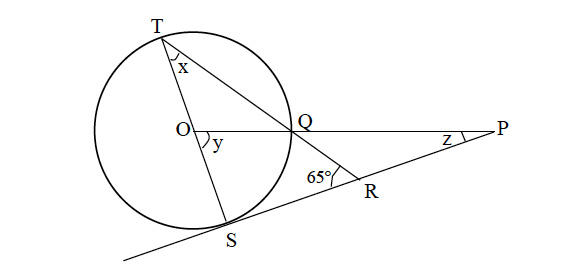In ΔRST, ∠s = 90° (∴ ST is a diameter)

∴ 𝑥 =180°− (90° + 65°); (angles of a triangle adds up to 180o)

= 25o

∠𝑦 = 2∠𝑥 = 2×25° = 50°

(angle of the centre is double the angle)

In the remaining circumference

𝑥 = 180°− (90° + 50°) = 40° (With at least one reason)

(angles of a triangle otherwise adds up to 180o)

### Question 4:

(a) Katrina opened a recurring deposit account with a Nationalised Bank for a period of 2 years. If the bank pays interest at the rate of 6% per annum and the monthly instalment is 1,000, find the:

(i) interest earned in 2 years.

(ii) matured value.

(b) Find the value of ‘K’ for which x = 3 is a solution of the quadratic equation,

(K + 2) x2 – Kx + 6 = 0.

Thus find the other root of the equation.

(c) Construct a regular hexagon of side 5 cm. Construct a circle circumscribing the hexagon. All traces of construction must be clearly shown.

(a) Instalment = 1000, number of months = 12 x 2 = 24, Rate = 6%

(i)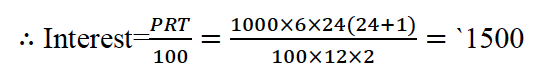(ii) Matured value = Total amount deposited + Interest

= 1000 x 24 + 1500 = 25,500

(b) (𝐾+2)𝑥2 − 𝐾𝑥 + 6 = 0, 𝑥=3 is a root of the equation

∴ (𝐾+2) 32 − 𝐾×3 + 6 = 0

9𝐾 + 18 − 3𝐾 + 6 = 0

∴ 6𝐾 = −24 𝑜𝑟 𝐾= −4

∴The equation is −2𝑥2 + 4𝑥 + 6 = 0

𝑥2 − 2𝑥 − 3 = 0

∴ (𝑥−3) (𝑥+1) = 0

∴ 𝑥 = 3, 𝑥 = −1

Hence the second root is -1

(c) One side and one 120o

Hexagon

Bisection of one side

Two sides bisection to locate centre and circumcircle

### Question 5:

(a) Use a graph paper for this question taking 1 cm = 1 unit along both the x and y axis:

(i) Plot the points A(0, 5), B(2, 5), C(5, 2), D(5, -2), E(2, -5) and F(0, -5).

(ii) Reflect the points B, C, D and E on the y-axis and name them respectively as B’, C’, D’ and E’.

(iii) Write the coordinates of B’, C’, D’ and E’.

(iv) Name the figure formed by B C D E E’ D’C’B’.

(v) Name a line of symmetry for the figure formed.

(b) Virat opened a Savings Bank account in a bank on 16th April 2010. His pass book shows the following entries:

 Date Particulars Withdrawal Deposit Balance April 12, 2010 By Cash – 2500 2500 April 28th By Cheque – 3000 5500 May 9th To Cheque 850 – 4650 May 15th By Cash – 1600 6250 May 24th To Cash 1000 – 5250 June 4th To Cash 500 – 4750 June 30th By Cheque – 2400 7150 July 3rd By Cash – 1800 8950

Calculate the interest Virat earned at the end of 31st July, 2010 at 4% per annum interest. What sum of money will he receive if he closes the account on 1st August, 2010?

(a) (i) Plotting 3 points correctly

(ii) Plotting 3 images correctly

(iii) Writing all coordinates correctly Bʹ(–2,5), Cʹ(–5,2), Dʹ(–5,–2), Eʹ(–2,–5)

(iv) Octagon

(v) y-axis or x-axis or any other correct line of symmetry B1 x 5

(b)

 Month Minimum Balance April 0 May 4650 June 4750 July 8950 18350

### Question 6:

(a) If a, b, c are in continued proportion, prove that

(a + b + c) (a – b + c) = a2 + b2 + c2

(b) In the given figure ABC is a triangle and BC is parallel to the y-axis. AB and AC intersects the y-axis at P and Q respectively.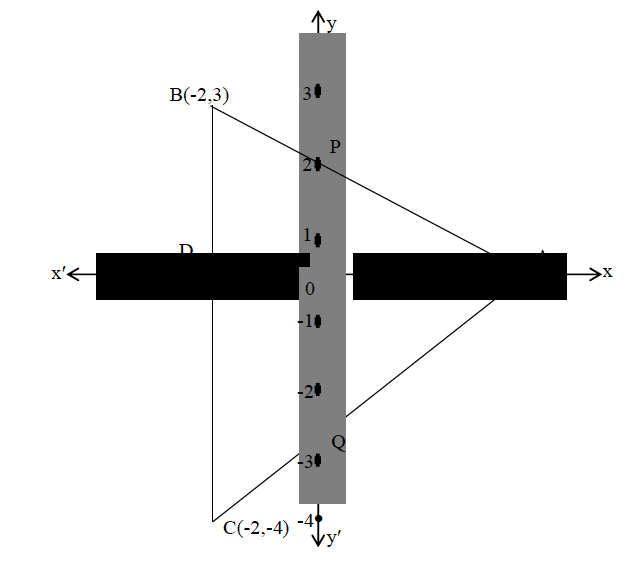(i) Write the coordinates of A.

(ii) Find the length of AB and AC.

(iii) Find the ratio in which Q divides AC.

(iv) Find the equation of the line AC.

(c) Calculate the mean of the following distribution:

 Class Interval 0-10 10-20 20-30 30-40 40-50 50-60 Frequency 8 5 12 35 24 16

(a) a,b,c are in continued proportion

∴a/b = b/c or b2 = ac

LHS = (a + b + c)(a − b + c) = a2 + c2 + 2ac − b2

= a2 + c2 + 2b2 − b2

= a2 + b2 + c2 = R.H.S. proved

(b)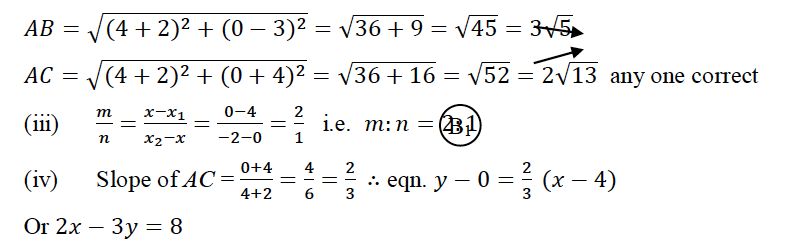(c)

 Class Interval Frequency X d = X – A fd 0-10 8 5 -30 -240 10-20 5 15 -20 -100 20-30 12 25 -10 -120 30-40 35 35 0 0 40-50 24 45 10 240 50-60 16 55 20 320 𝜀𝑓 = 100 𝜀𝑓d = 100

Mean = 𝐴 + (𝜀𝑓d/𝜀𝑓) = 35 + (100/100) = 36

### Question 7:

(a) Two solid spheres of radii 2 cm and 4 cm are melted and recast into a cone of height 8 cm. Find the radius of the cone so formed.

(b) Find ‘a’ if the two polynomials ax3 + 3x2 – 9 and 2x3 + 4x + a, leaves the same remainder when divided by x+3.

(c)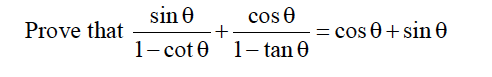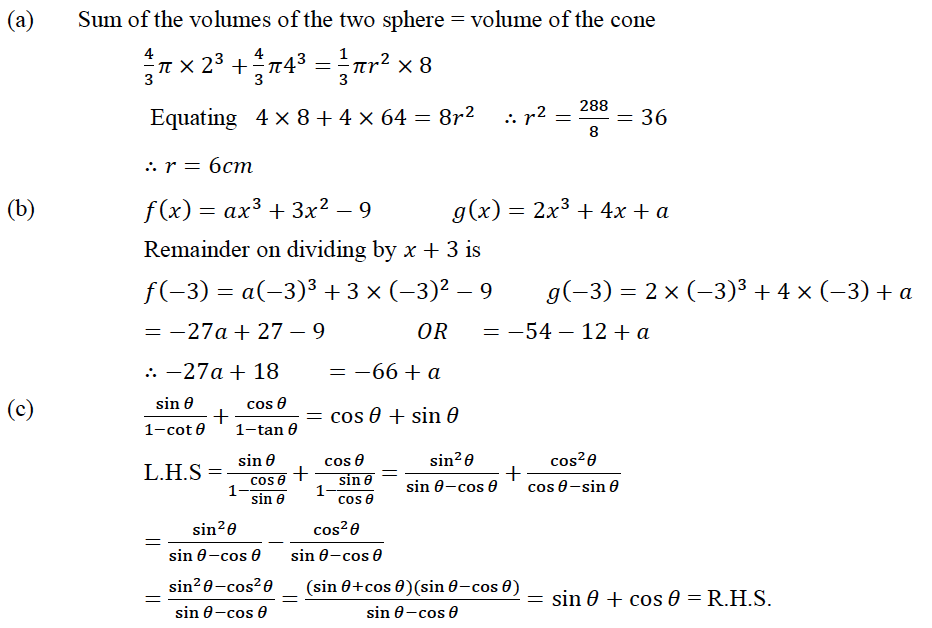### Question 8:

(a) AB and CD are two chords of a circle intersecting at P. Prove that AP × PB = CP × PD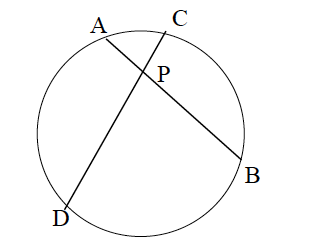(b) A bag contains 5 white balls, 6 red balls and 9 green balls. A ball is drawn at random from the bag. Find the probability that the ball drawn is:

(i) a green ball

(ii) a white or a red ball

(iii) is neither a green ball nor a white ball.

(c) Rohit invested ₹9,600 on ₹100 shares at ₹20 premium paying 8% dividend. Rohit sold the shares when the price rose to ₹160. He invested the proceeds (excluding dividend) in 10% ₹50 shares at ₹40. Find the:

(i) original number of shares.

(ii) sale proceeds.

(iii) new number of shares.

(iv) change in the two dividends.

(a) In the two triangles APD and CPB∠A = ∠C (angles in the same segment)

∠D = ∠B

∠APD = ∠CPD

∴ ΔAPD ~ ΔCPB (AAA)

Hence AP/CP = PD/PB or AP x PB = CP x PD

(b) 5 white balls, 6 red balls and 9 green balls

(i) There are 9 green balls and 5 + 6 + 9 = 20 balls altogether

∴ P (a green ball) = 9/20

(ii) There are 5 white balls and 6 red balls and their sum is 11

Total number of balls = 20

∴ P (a white or red ball) = 11/20

(iii) Neither green or white means probability of a red ball. There are 6 red balls

Total number of balls = 20

∴ P(neither green nor white) = 6/20 = 3/10

(c) Total investment = ₹9600 N.V. = ₹100 M.V. = 100 + 20 = ₹120, rate of dividend = 8%.

(i) ∴ original number of shares = 9600/120 = 80

(ii) Sale proceeds = 80 x 160 = ₹12800

(iii) The new number of shares = 12800 ÷ 40 = 320

(iv) Dividend from the original shares = 80 x 100 x 8 100 = ₹640

Dividend from the new shares = 320 x 50 x 10 100 = ₹1600

∴ Change in the two dividends = ₹1600 – ₹640 = `960

### Question 9:

(a) The horizontal distance between two towers is 120m. The angle of elevation of the top and angle of depression of the bottom of the first tower as observed from the second tower is 30° and 24° respectively.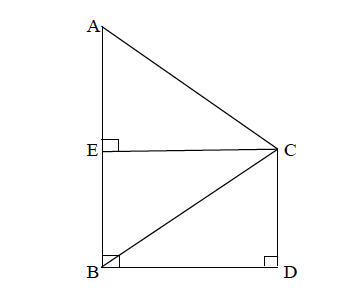Find the height of the two towers. Give your answer correctly to 3 significant figures.

(b) The weight of 50 workers is given below:

 Weight in Kg 50-60 60-70 70-80 80-90 90-100 100-110 110-120 No. of Workers 4 7 11 14 6 5 3

(a)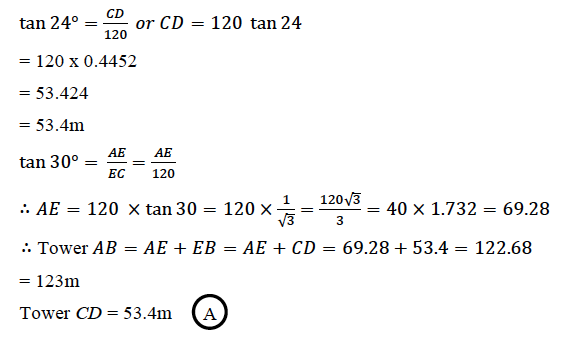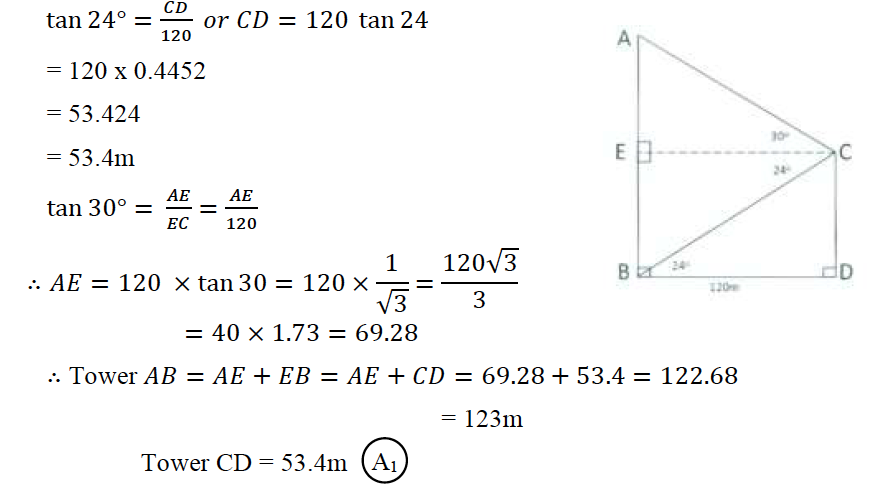(b)

 Weight in Kg Number of Persons C.f. 50-60 4 4 60-70 7 11 70-80 11 22 80-90 14 36 90-100 6 42 100-110 5 47 110-120 3 50

S-curve plotted with respect to upper boundaries and C.f.

(i) Q1 position = (1/4) × 50 = 12.5

∴ Q1 = 71 kg (±1)

Q3 position = (3/4) × 50 = 37.5

∴ Q3 = 93 kg (±1)

Correct axis and perpendiculars dropped for result

(ii) Number of persons who are overweight is equal to

50 – 38.5 = 11.5 (±1) = 11 or 12 approximately

### Question 10:

(a) A wholesaler buys a TV from the manufacturer for ₹25,000. He marks the price of the TV 20% above his cost price and sells it to a retailer at a 10% discount on the marked price. If the rate of VAT is 8%, find the:

(i) marked price.

(ii) retailer’s cost price inclusive of tax.

(iii) VAT paid by the wholesaler.

(b)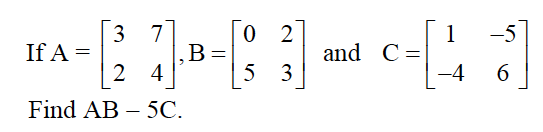(c) ABC is a right angled triangle with ABC = 90°. D is any point on AB and DE is perpendicular to AC. Prove that: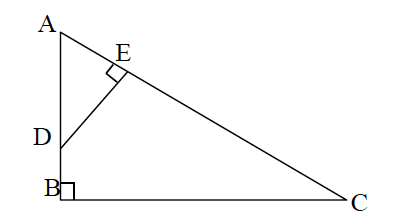(ii) If AC = 13 cm, BC = 5 cm and AE = 4 cm. Find DE and AD.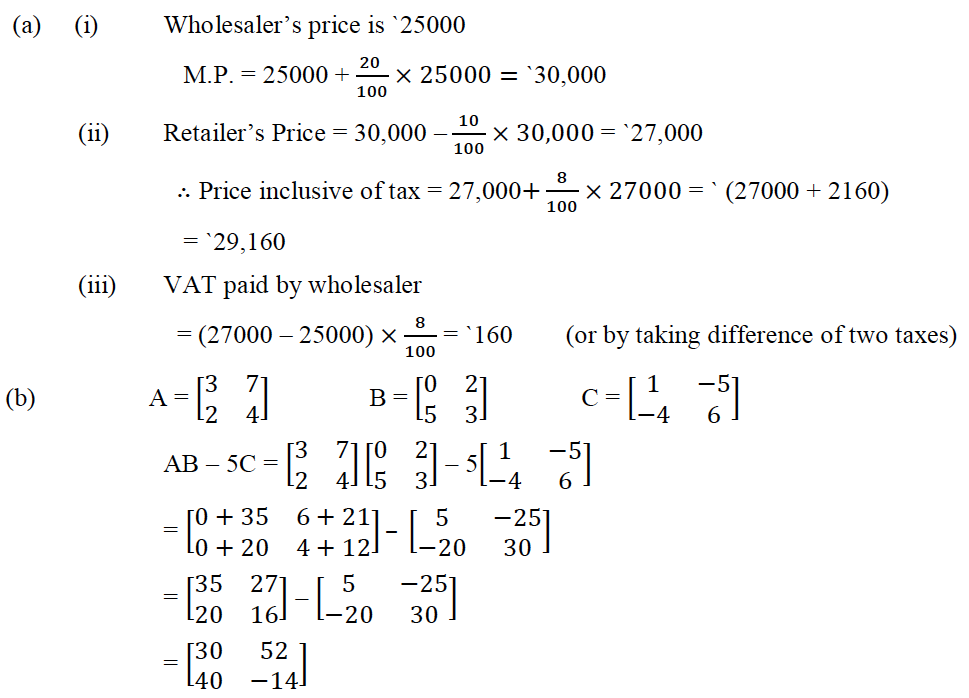### Question 11:

(a) Sum of two natural numbers is 8 and the difference of their reciprocal is 2/15. Find the numbers.

(b) Given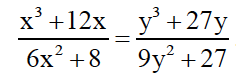Using componendo and dividendo find x: y.

(c) Construct a triangle ABC with AB = 5.5. cm, AC = 6 cm and BAC = 105°. Hence:

(i) Construct the locus of points equidistant from BA and BC.

(ii) Construct the locus of points equidistant from B and C.

(iii) Mark the point which satisfies the above two loci as P. Measure and write the length of the PC.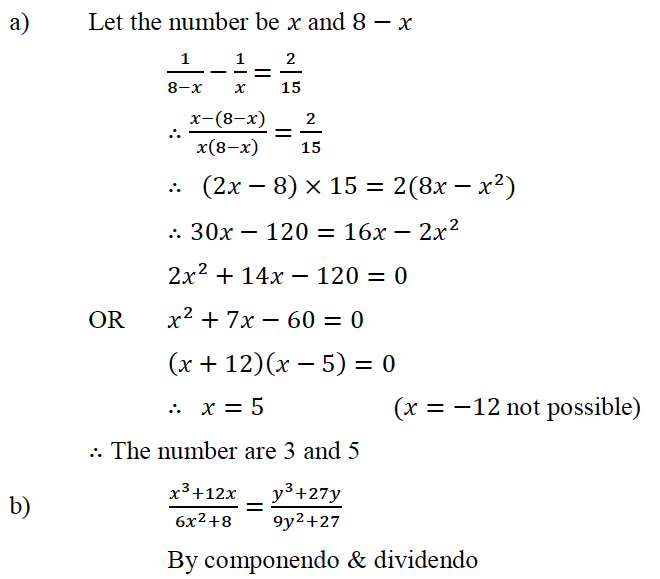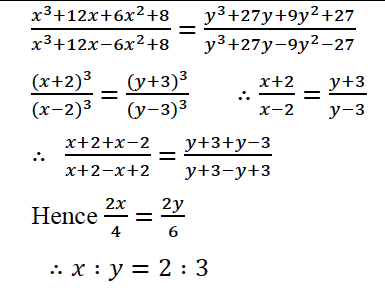(c) ∠BAC = 105°

ΔABC

Bisector ∠B

Bisector of BC or

PC = 5cm

The ICSE Class 10 Maths Question Paper Solution 2015 must have helped students with their exam preparation. The answers in the Solution pdf have provided an in-depth to 2015 questions and the way answers to be written to score high marks in the board exam. Apart from the 2015 paper solution, students can find the answers to other papers of ICSE Class 10 Previous Years Questions by clicking here. Happy Learning and stay tuned to BYJU’S for the latest update on ICSE/CBSE/State Boards/Competitive exams. Also, don’t forget to download the BYJU’S App.